Exit Intent

## Basic Electrical Engineering

1.It is preferable to connect bulbs in series or in parallel?
a) Series
b) Parallel
c) Both series and parallel
d) Neither series nor parallel
Explanation: Bulbs are connected in parallel so that even if one of the bulbs blow out, the others continue to get a current supply.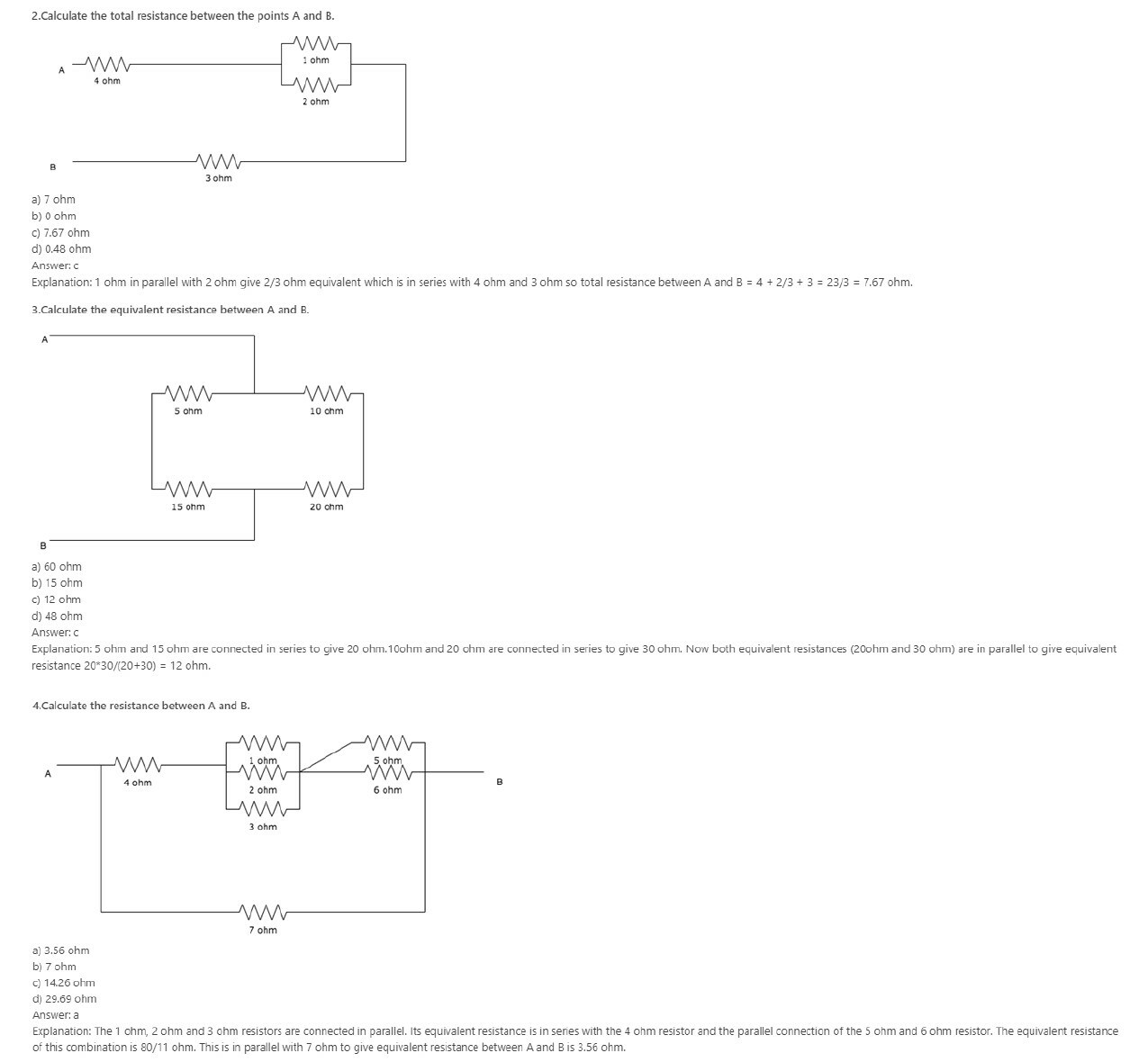5.Batteries are generally connected in______
a) Series
b) Parallel
c) Either series or parallel
d) Neither series nor parallel
Explanation: Batteries are generally connected in series so that we can obtain the desired voltage since voltages add up once they are connected in series.

6.In a _________ circuit, the total resistance is greater than the largest resistance in the circuit.
a) Series
b) Parallel

c) Either series or parallel

d) Neither series nor parallel

Explanation: In series circuits, the total resistance is the sum of all the resistance in the circuit, hence the total is greater than the largest resistance.

7.In a ____________ circuit, the total resistance is smaller than the smallest resistance in the circuit.

a) Series

b) Parallel

c) Either series or parallel

d) Neither series nor parallel

Explanation: in a parallel circuit, the equivalent resistance=1/sum of the reciprocals of all the resistances in the circuit. Hence it is smaller than the smallest resistance in the circuit.

8.Which is the most cost efficient connection?

a) Series

b) Parallel

c) Either series or parallel

d) Neither series nor parallel

Explanation: The advantage of series-connections is that they share the supply voltage, hence cheap low voltage appliances may be used.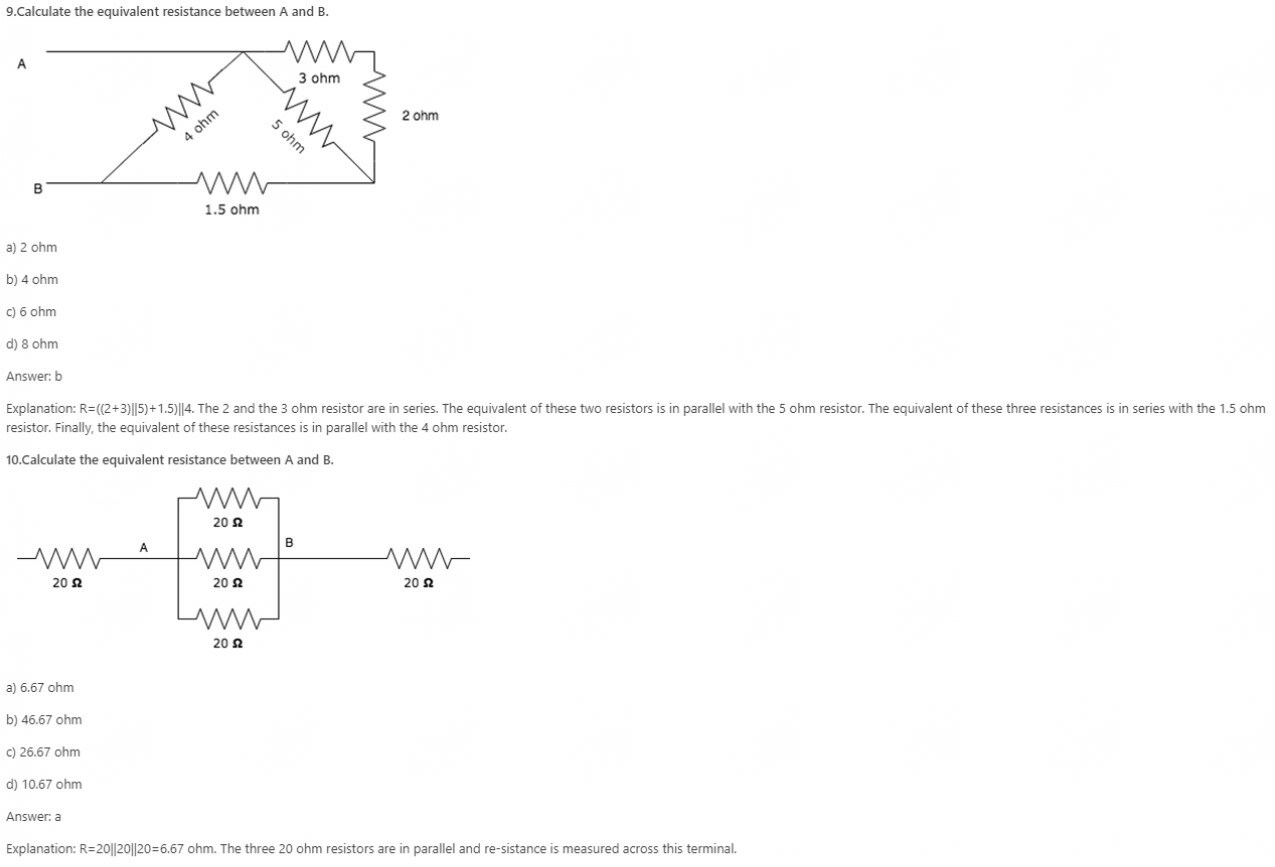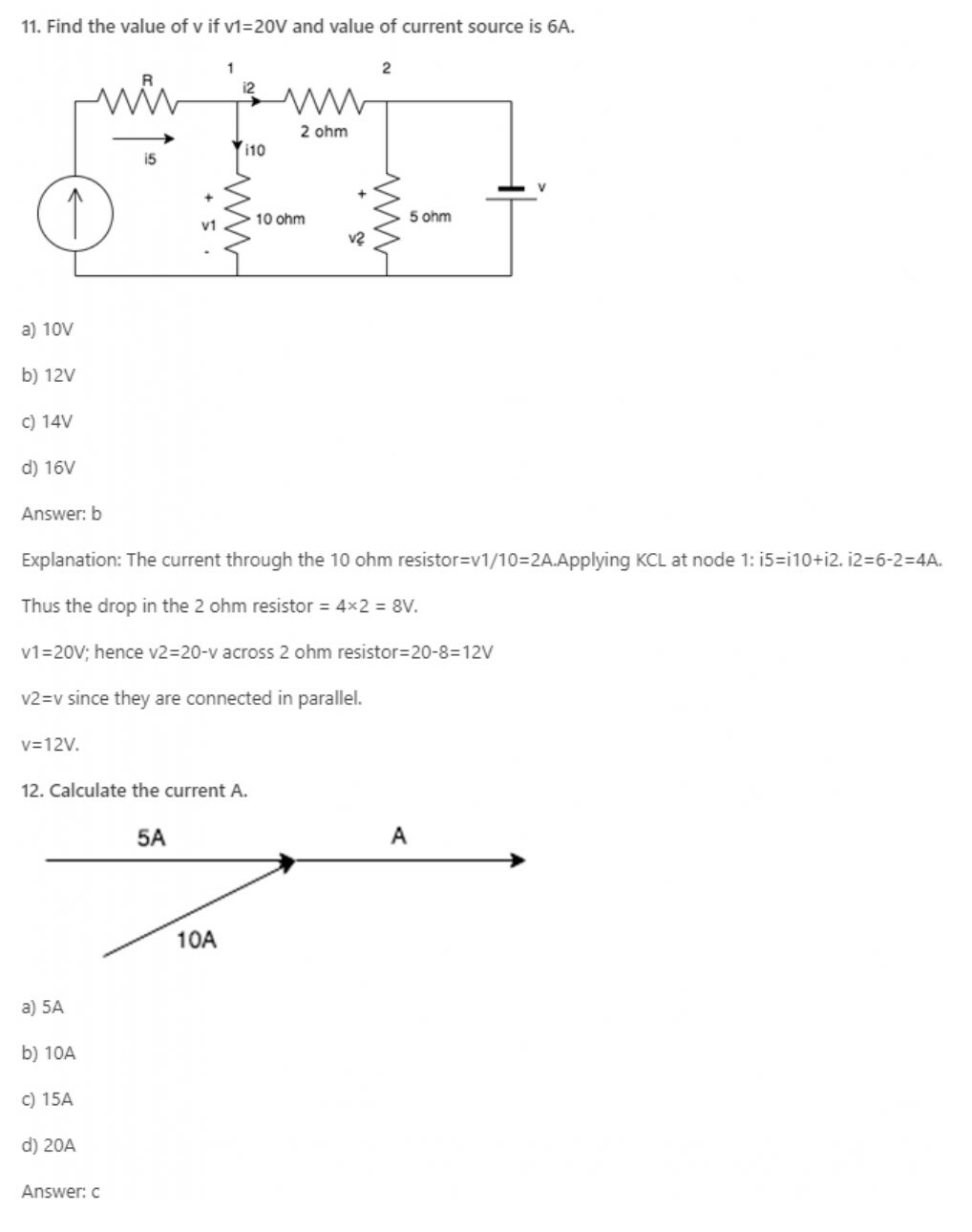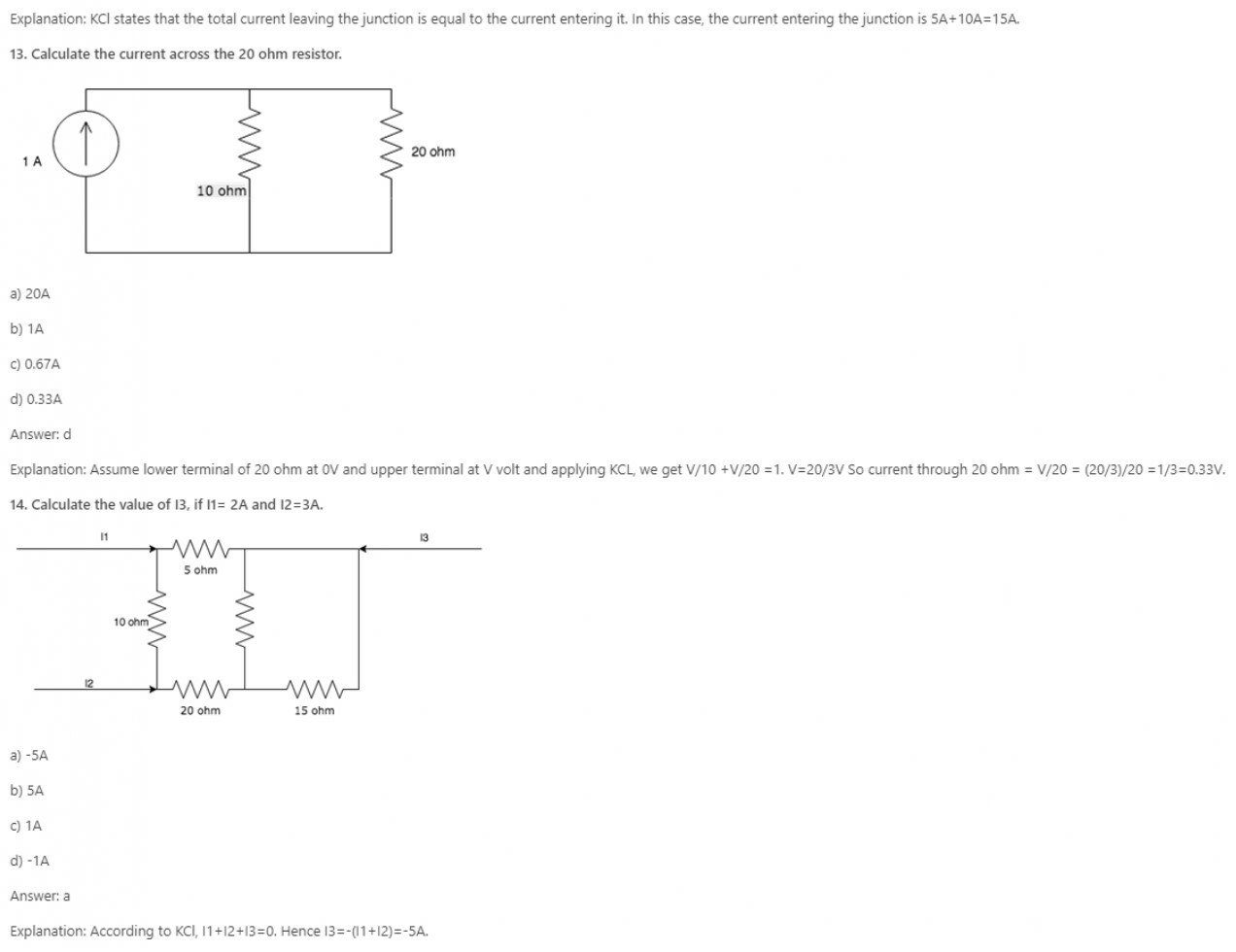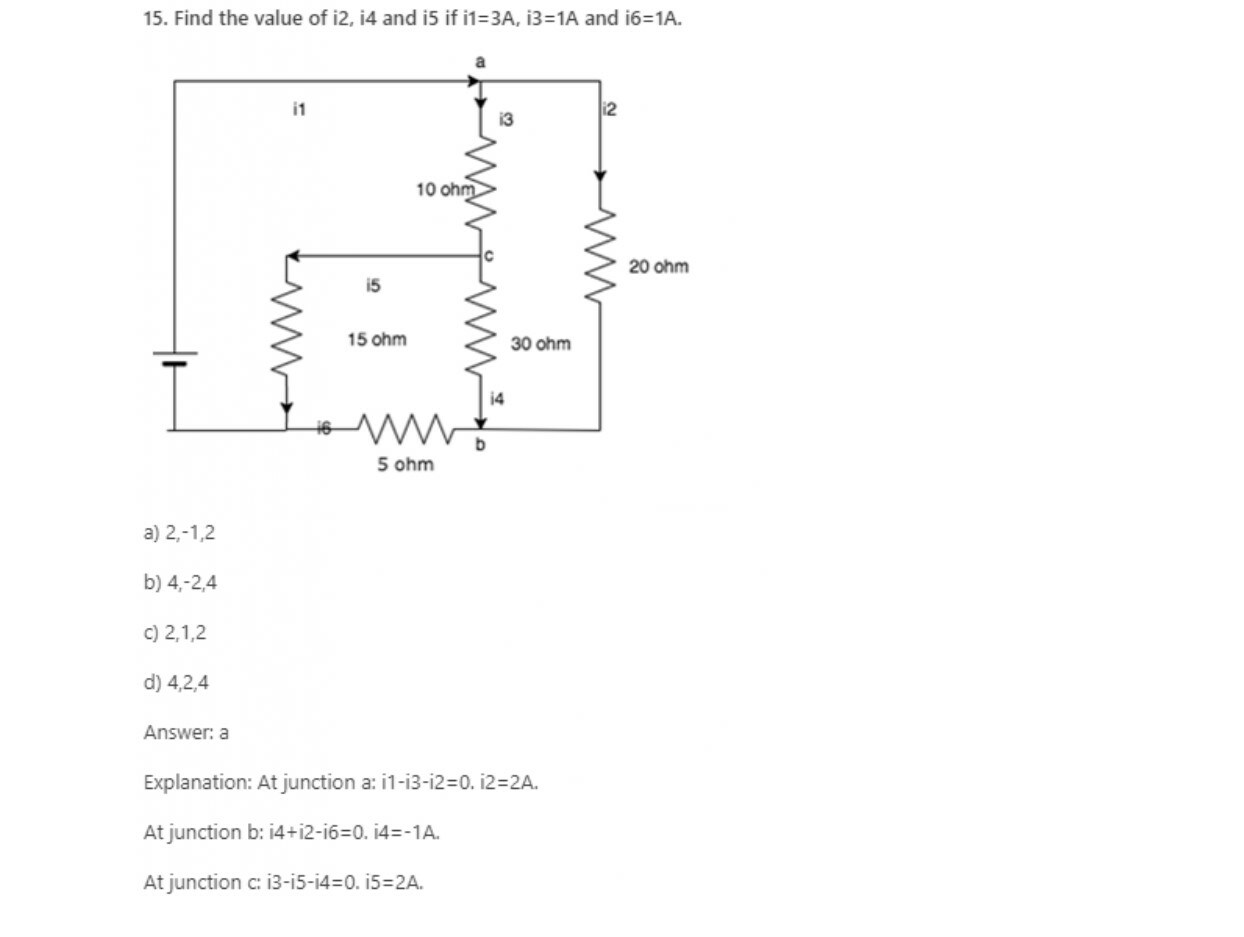16. What is the value of current if a 50C charge flows in a conductor over a period of 5 seconds?

a) 5A

b) 10A

c) 15A

d) 20A

Explanation: Current=Charge/Time. Here charge = 50c and time = 5seconds, so current = 50/5 = 10A.

17. KCL deals with the conservation of?

a) Momentum

b) Mass

c) Potential Energy

d) Charge

Explanation: KCL states that the amount of charge entering a junction is equal to the amount of charge leaving it, hence it is the conservation of charge.

18. KCL is applied at _________

a) Loop

b) Node

c) Both loop and node

d) Neither loop nor node

Explanation: KCL states that the amount of charge leaving a node is equal to the amount of charge entering it, hence it is applied at nodes.

Python Programming for Complete Beginners

19. KCL can be applied for __________

a) Planar networks

b) Non-planar networks

c) Both planar and non-planar

d) Neither planar nor non-planar

Explanation: KCL is applied for different nodes of a network whether it is planar or non-planar.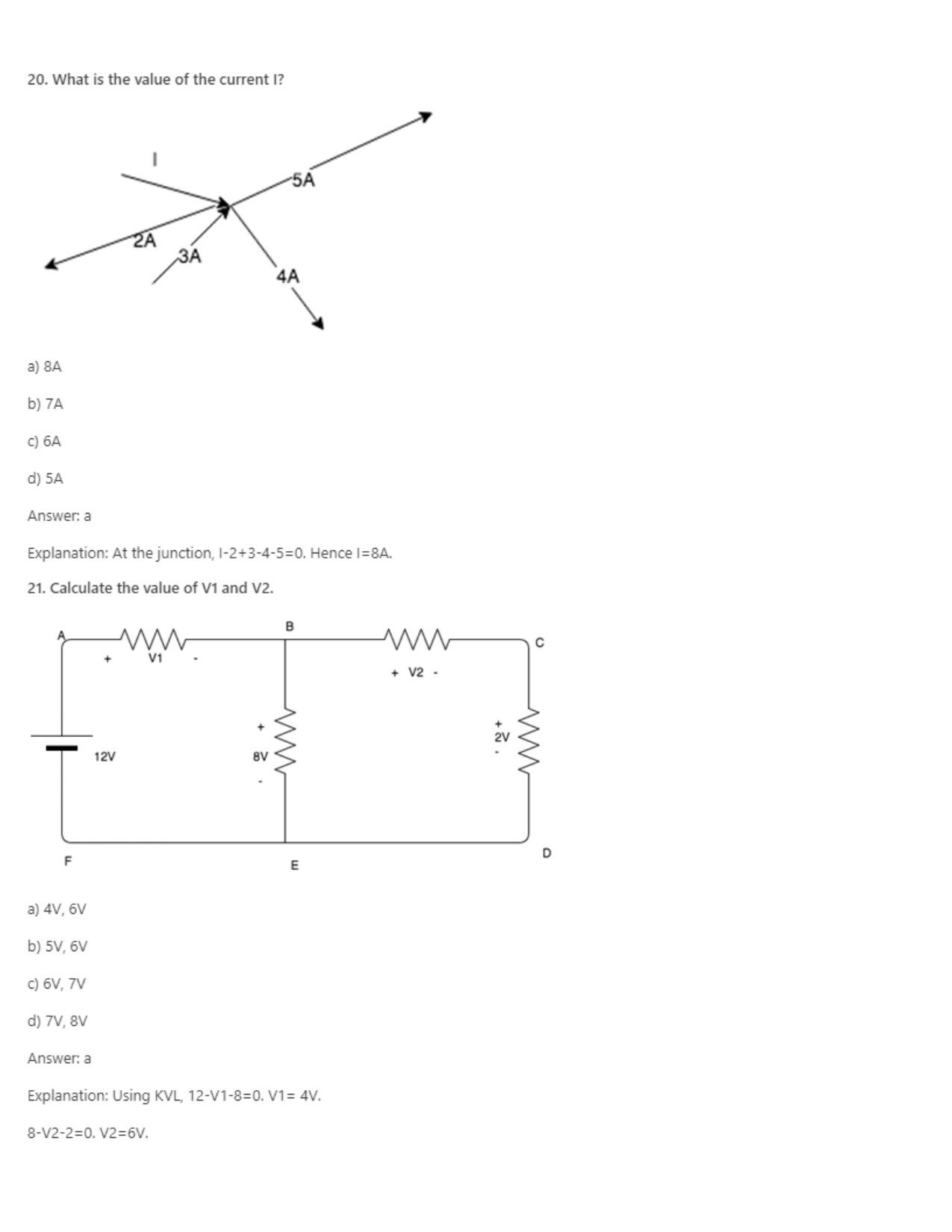22. KVL deals with the conservation of?

a) Mass

b) Momentum

c) Charge

d) Energy

Explanation: KVL states that the sum of the potential energy and taken with the right sign is equal to zero, hence it is the conservation of energy since energy doesn’t enter or leave the system.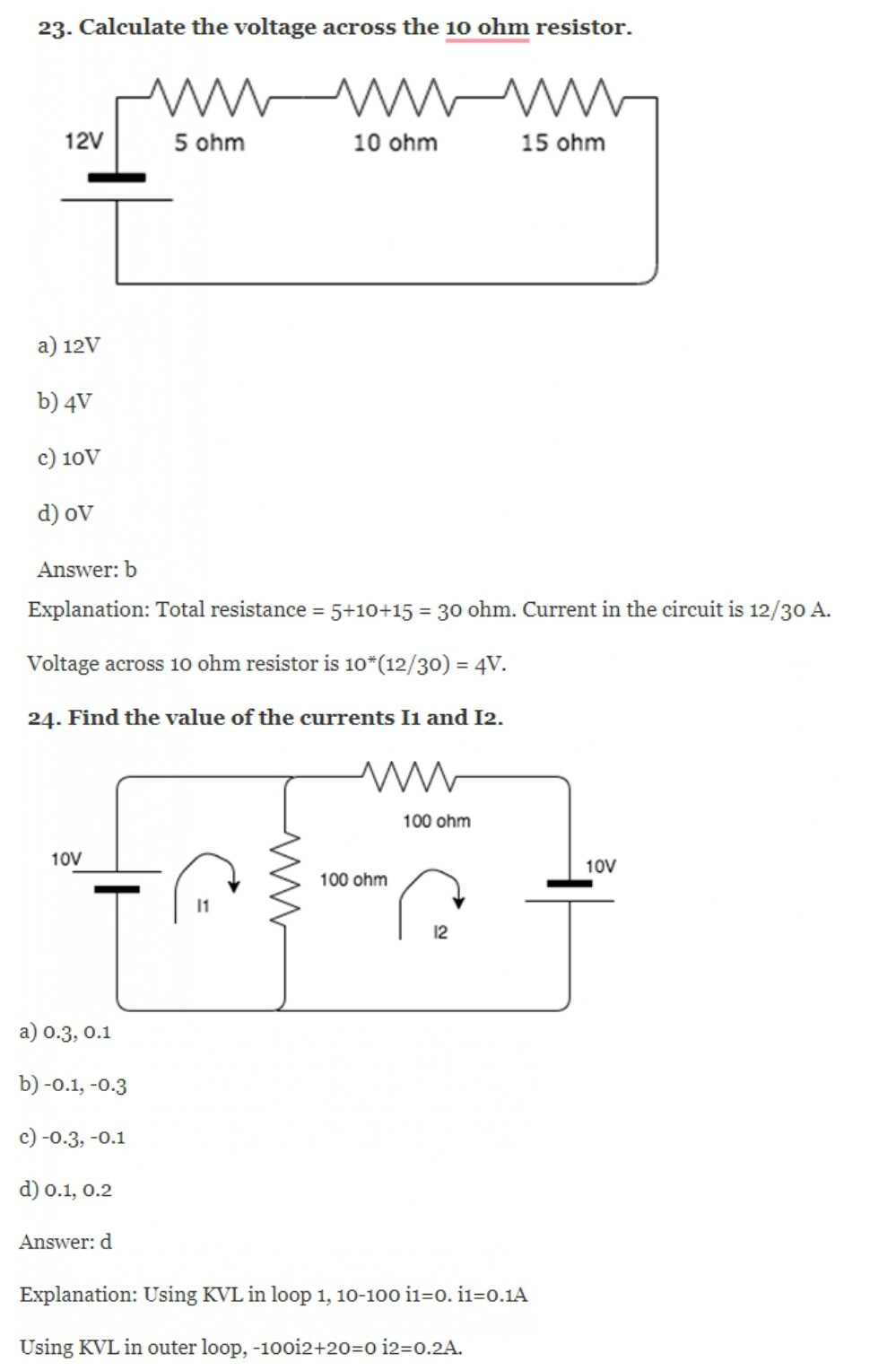Get Engineering Viva Question with Answers

25. The sum of the voltages over any closed loop is equal to __________

a) 0V

b) Infinity

c) 1V

d) 2V

Explanation: According to KVL, the sum of the voltage over any closed loop is equal to 0.

26. What is the basic law that has to be followed in order to analyze the circuit?

a) Newton’s laws

c) Ampere’s laws

d) Kirchhoff’s law

Explanation: Kirchhoff’s laws, namely Kirchhoff’s Current Law and Kirchhoff’s Voltage law are the basic laws in order to analyze a circuit.

27. Every____________ is a ____________ but every __________ is not a __________

a) Mesh, loop, loop, mesh

b) Loop, mesh, mesh, loop

c) Loop, mesh, loop, mesh

d) Mesh, loop, mesh, loop

Explanation: According to Kirchhoff’s Voltage Law, Every mesh is a loop but every loop is not a mesh. Mesh is a special case of loop which is planar.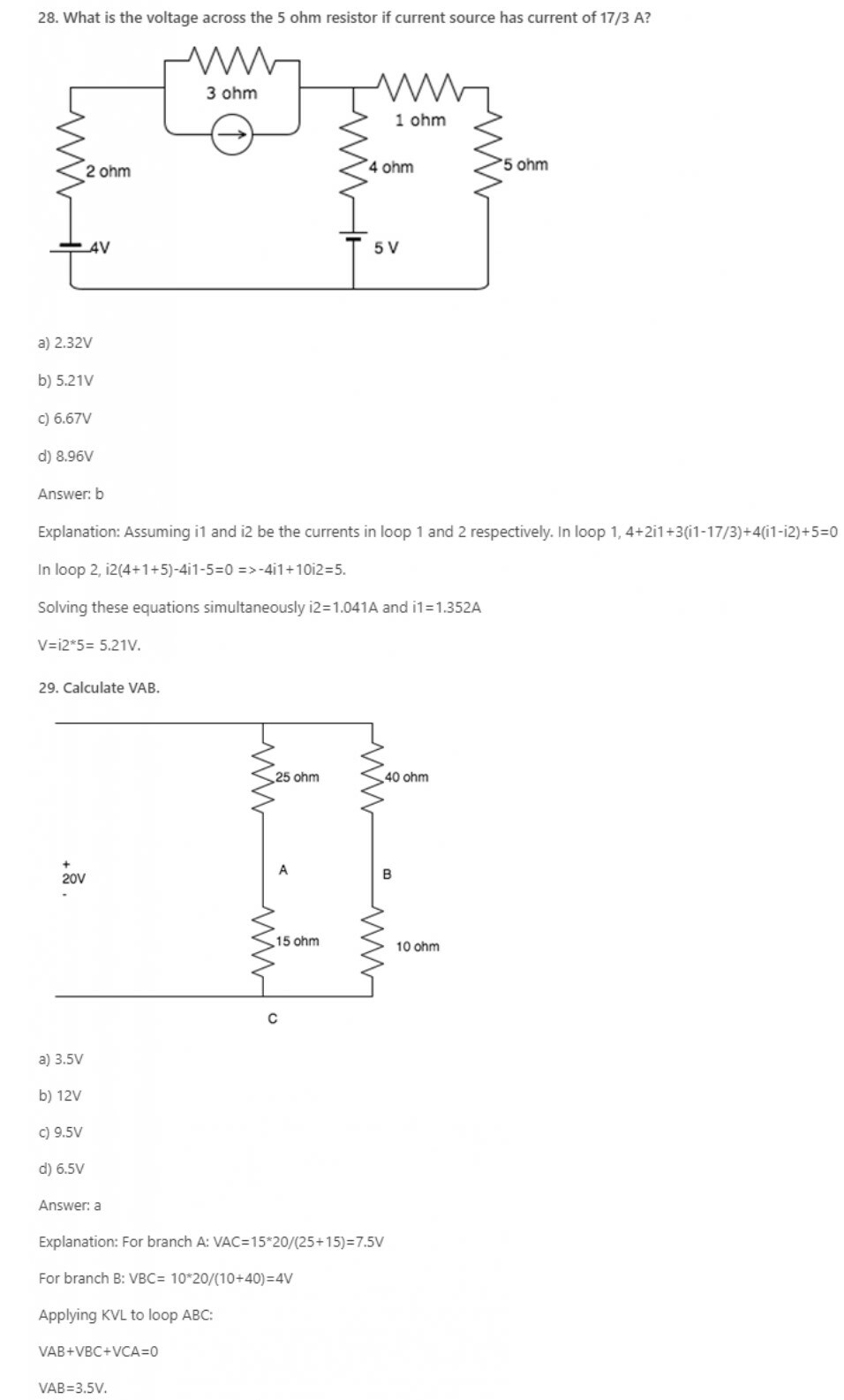Python Programming for Complete Beginners

30. KVL is applied in ____________

a) Mesh analysis

b) Nodal analysis

c) Both mesh and nodal

d) Neither mesh nor nodal

Explanation: Mesh analysis helps us to utilize the different voltages in the circuit as well as the IR products in the circuit which is nothing but KVL.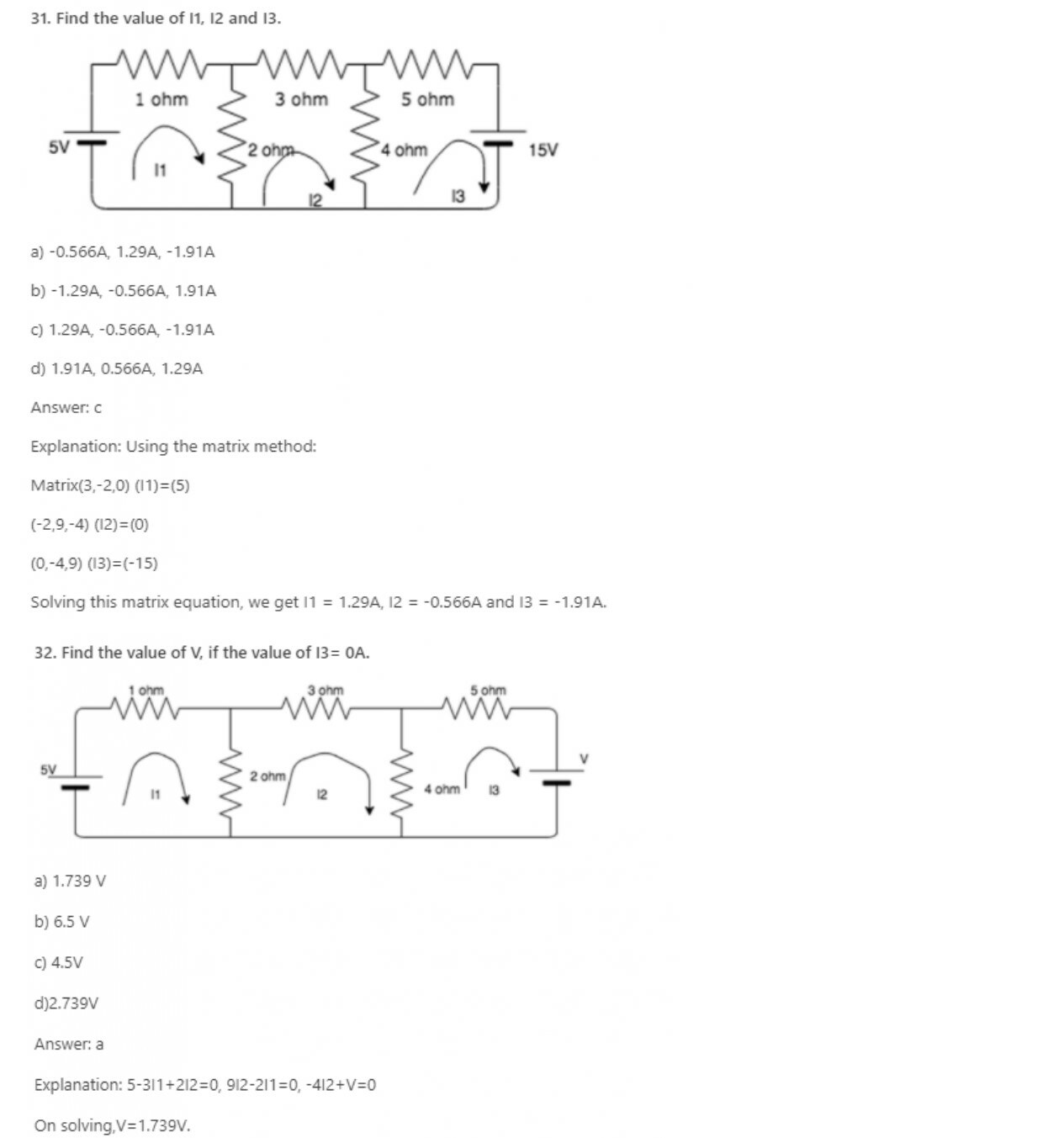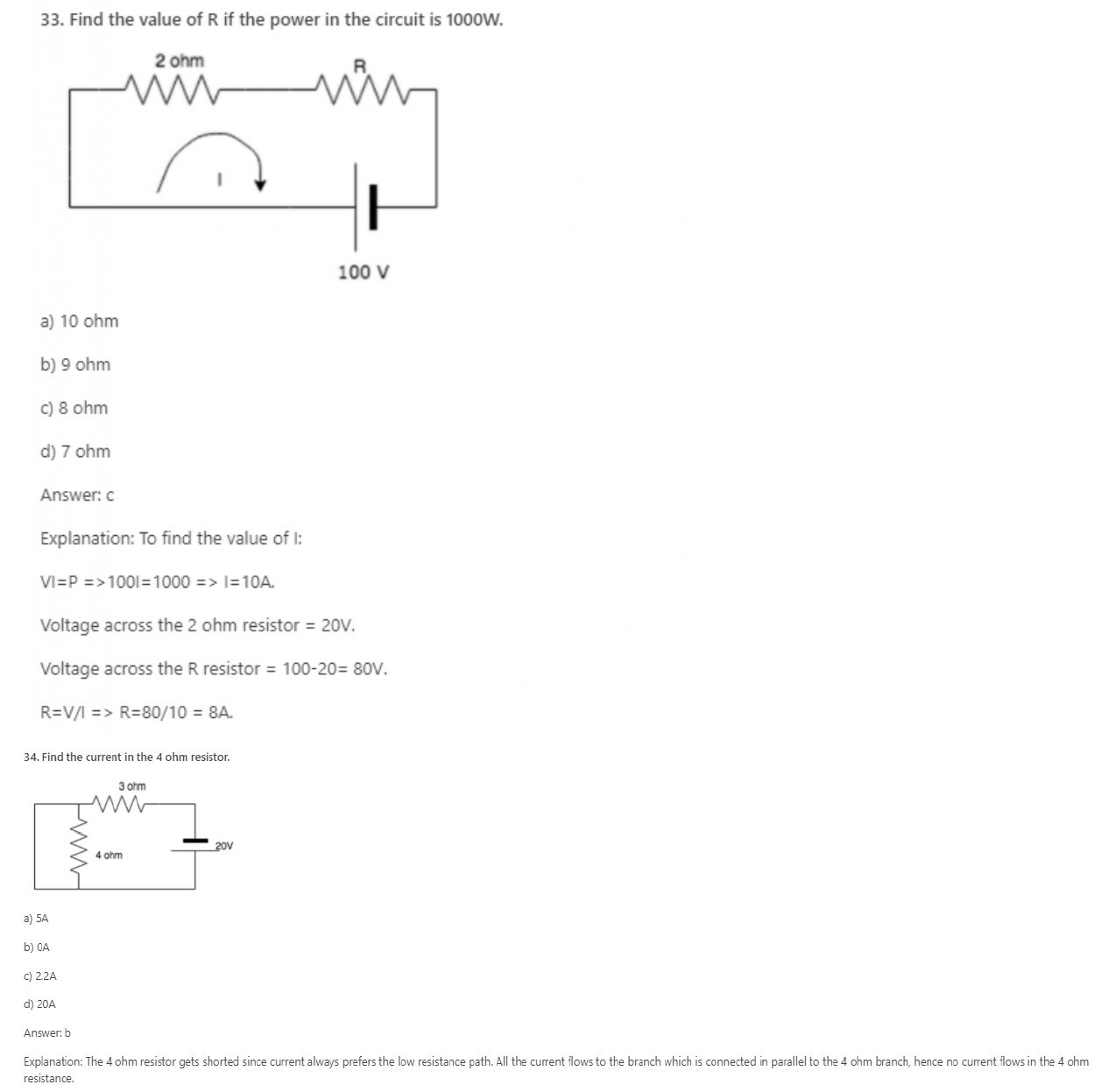35. Nodal analysis is generally used to determine______

a) Voltage

b) Current

c) Resistance

d) Power

Explanation: Nodal analysis uses Kirchhoff’s Current Law to find all the node voltages. Hence it is a method used to determine the voltage.

36. Mesh analysis is generally used to determine_________

a) Voltage

b) Current

c) Resistance

d) Power

Explanation: Mesh analysis uses Kirchhoff’s Voltage Law to find all the mesh currents. Hence it is a method used to determine current.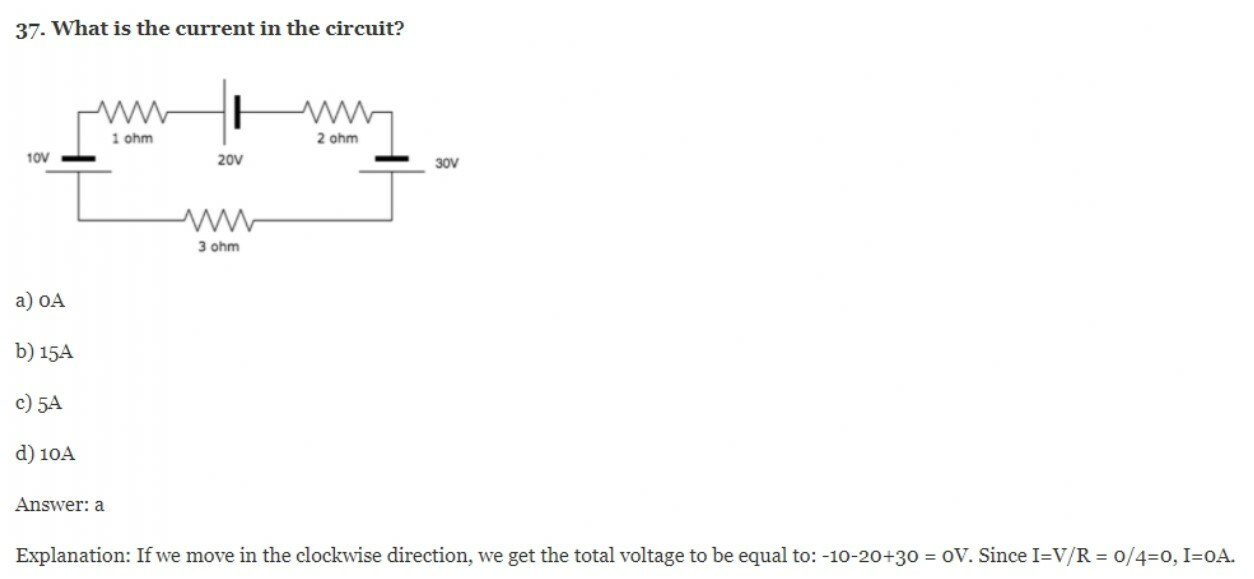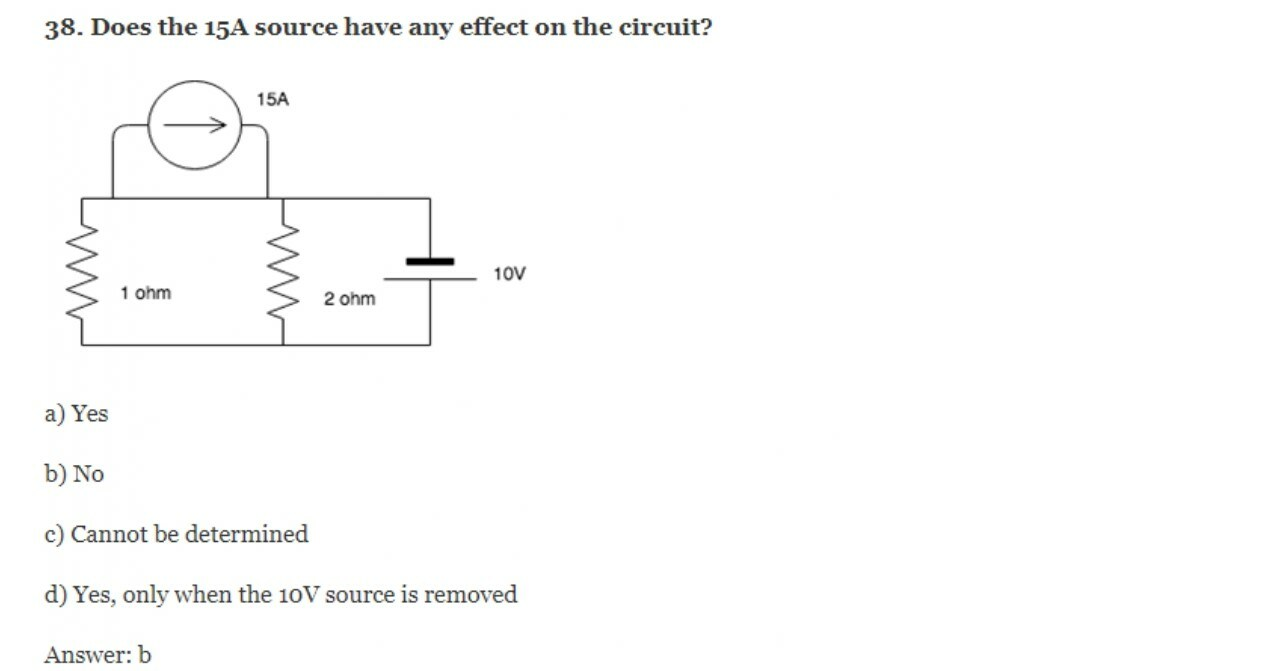Explanation: The 15A current source has a lower resistance path associated with it and hence it keeps moving in that particular loop. It does not leave that loop and enter the circuit, hence the circuit is not affected by it.

39. KVL is associated with____________

a) Mesh analysis

b) Nodal analysis

c) Both mesh and nodal

d) Neither mesh nor nodal

Explanation: KVL employs mesh analysis to find the different mesh currents by finding the IR products in each mesh.

Python Programming for Complete Beginners

40. KCL is associated with_________

a) Mesh analysis

b) Nodal analysis

c) Both mesh and nodal

d) Neither mesh nor nodal

Explanation: KCL employs nodal analysis to find the different node voltages by finding the value if a current in each branch.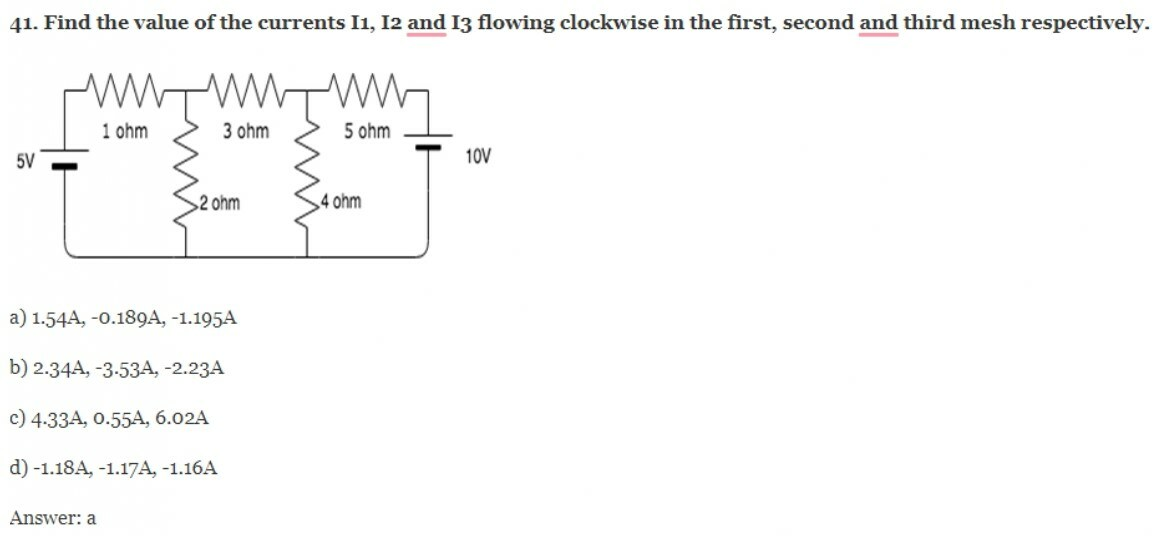Explanation: The three mesh equations are:

-3I1+2I2-5=0

2I1-9I2+4I3=0

4I2-9I3-10=0

Solving the equations, we get I1= 1.54A, I2=-0.189 and I3= -1.195A.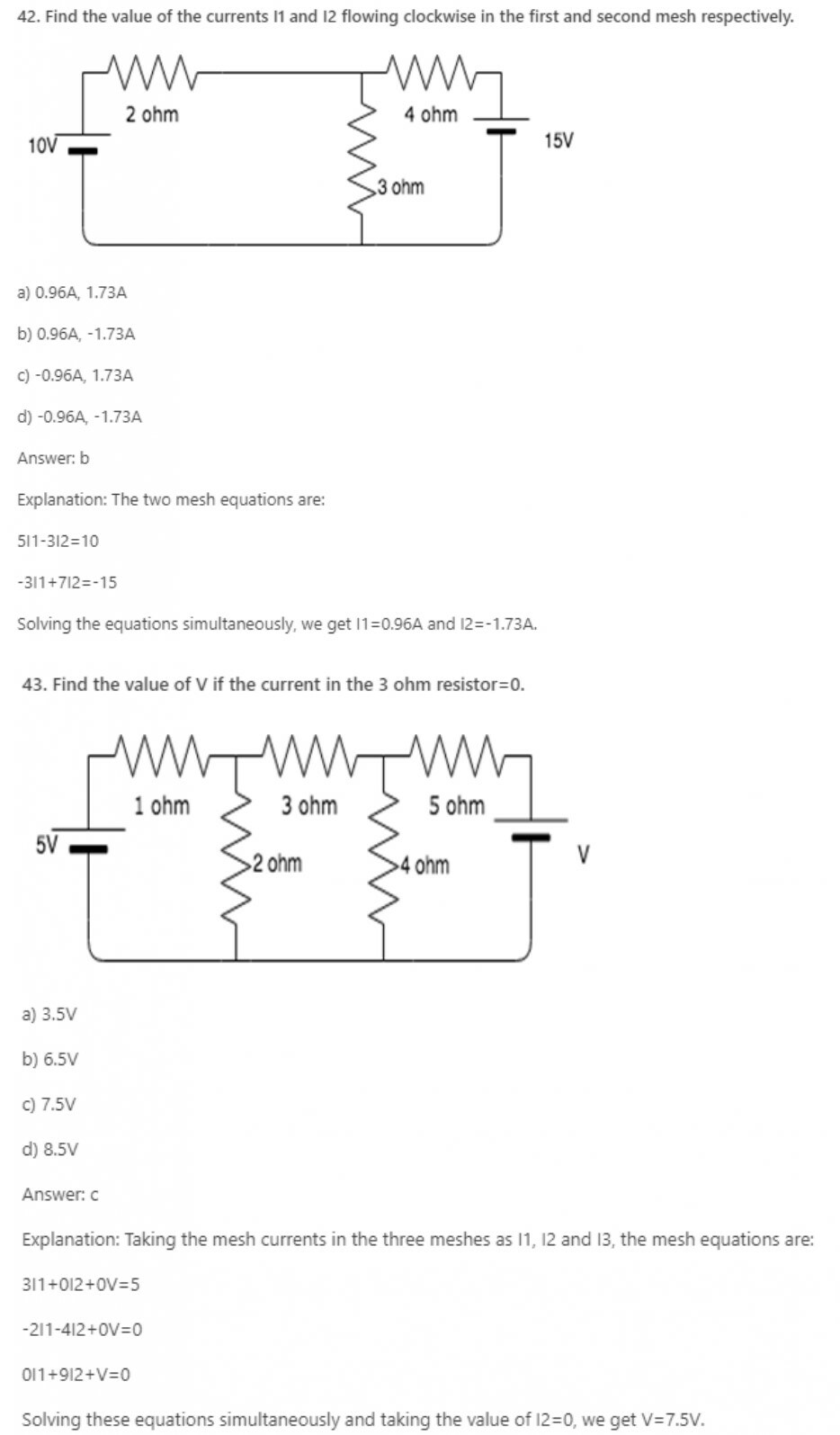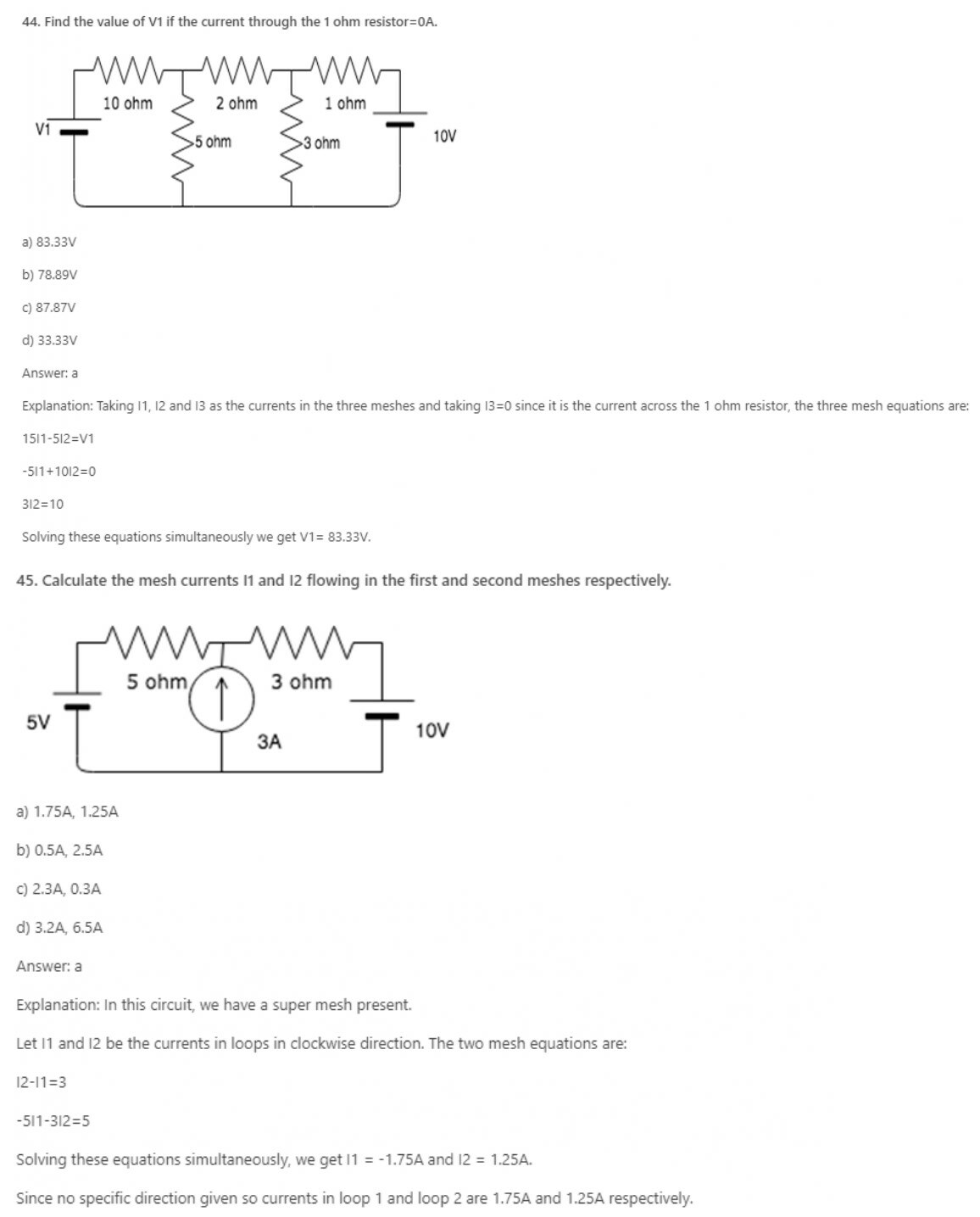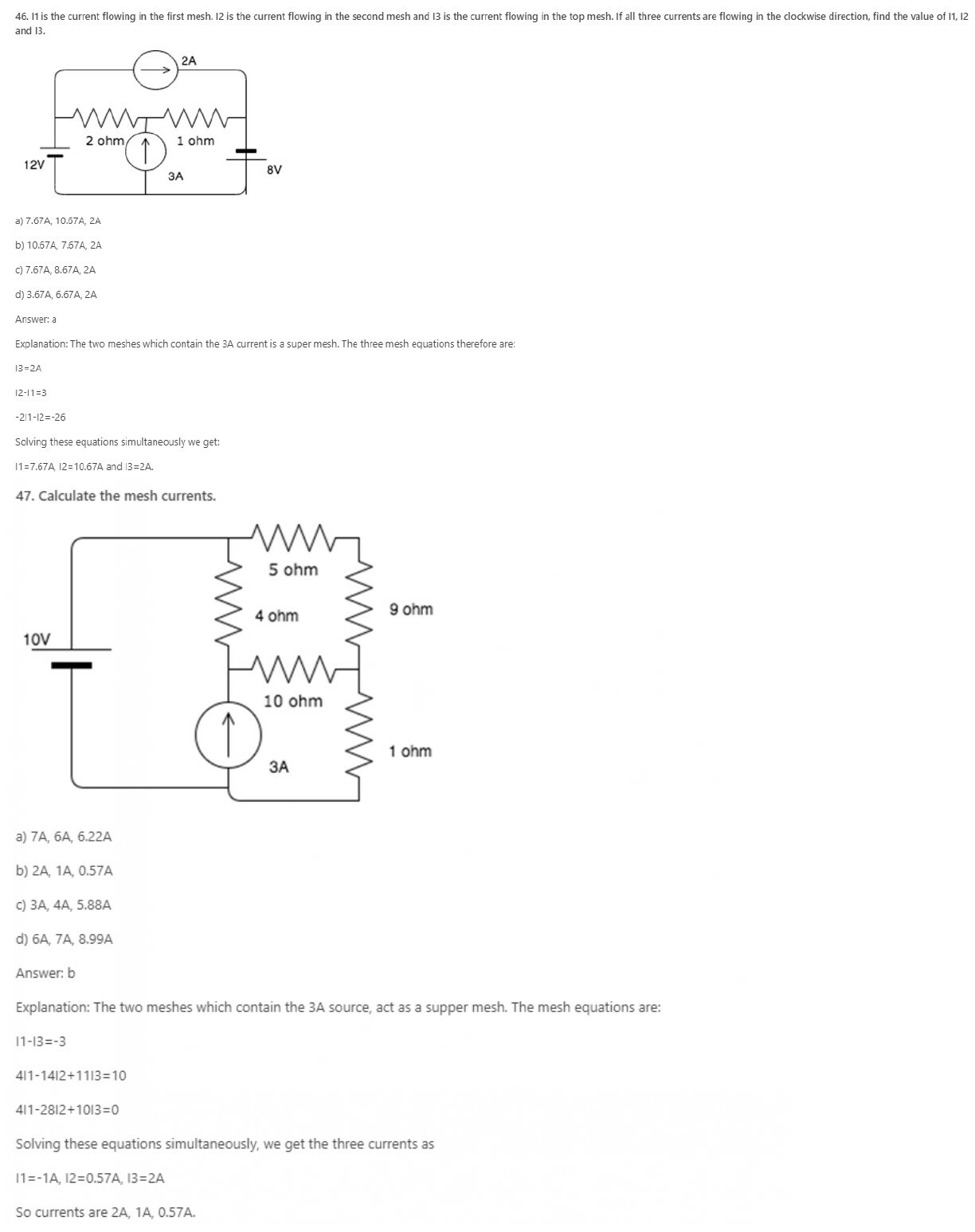48. Mesh analysis employs the method of ___________

a) KVL

b) KCL

c) Both KVL and KCL

d) Neither KVL nor KCL

Explanation: KVL employs mesh analysis to find the different mesh currents by finding the IR products in each mesh.

49. Mesh analysis is generally used to determine _________

a) Voltage

b) Current

c) Resistance

d) Power

Explanation: Mesh analysis uses Kirchhoff’s Voltage Law to find all the mesh currents. Hence it is a method used to determine current.

Python Programming for Complete Beginners

50. Mesh analysis can be used for __________

a) Planar circuits

b) Non-planar circuits

c) Both planar and non-planar circuits

d) Neither planar nor non-planar circuits

Explanation: If the circuit is not planar, the meshes are not clearly defined. In planar circuits, it is easy to draw the meshes hence the meshes are clearly defined.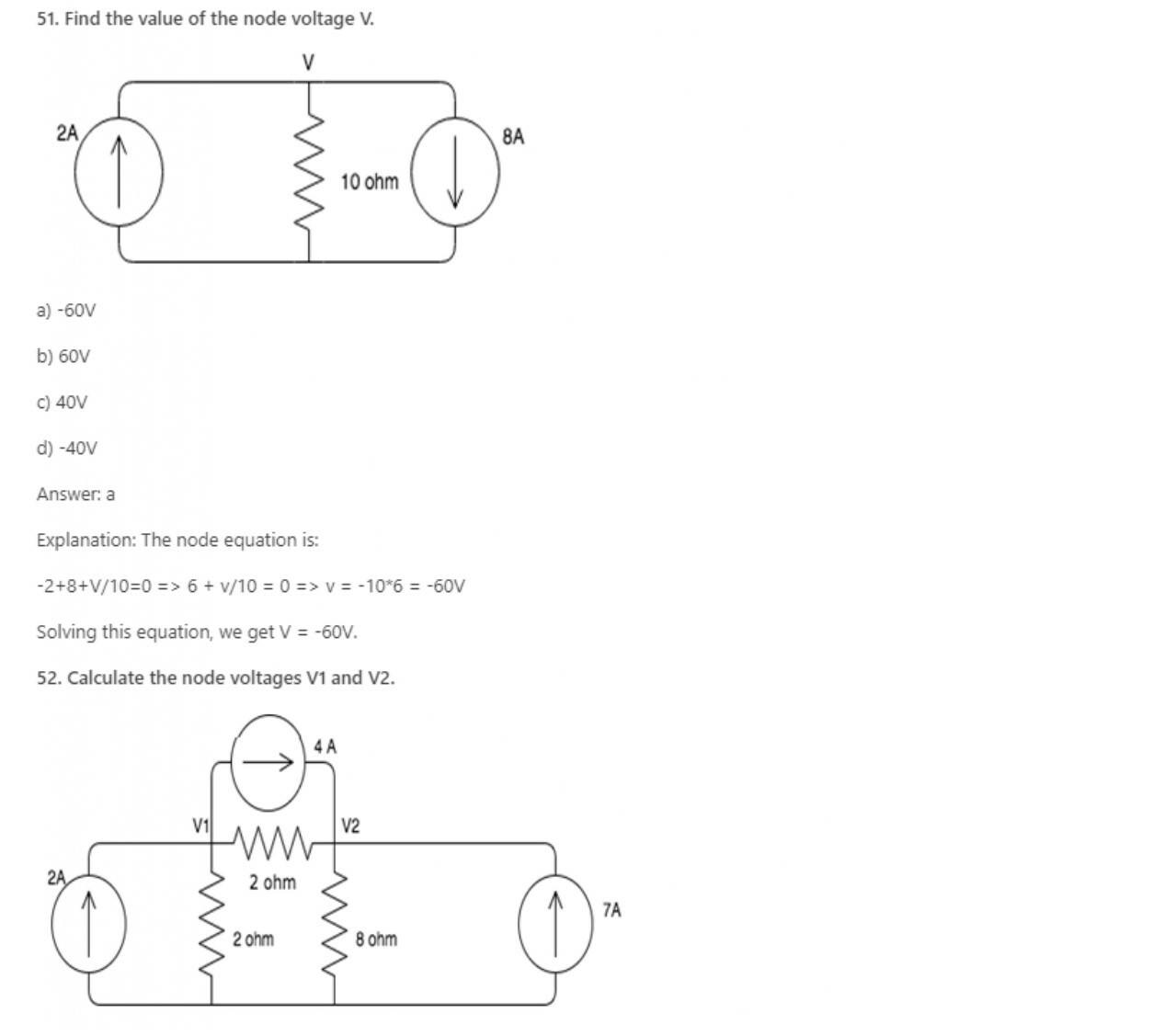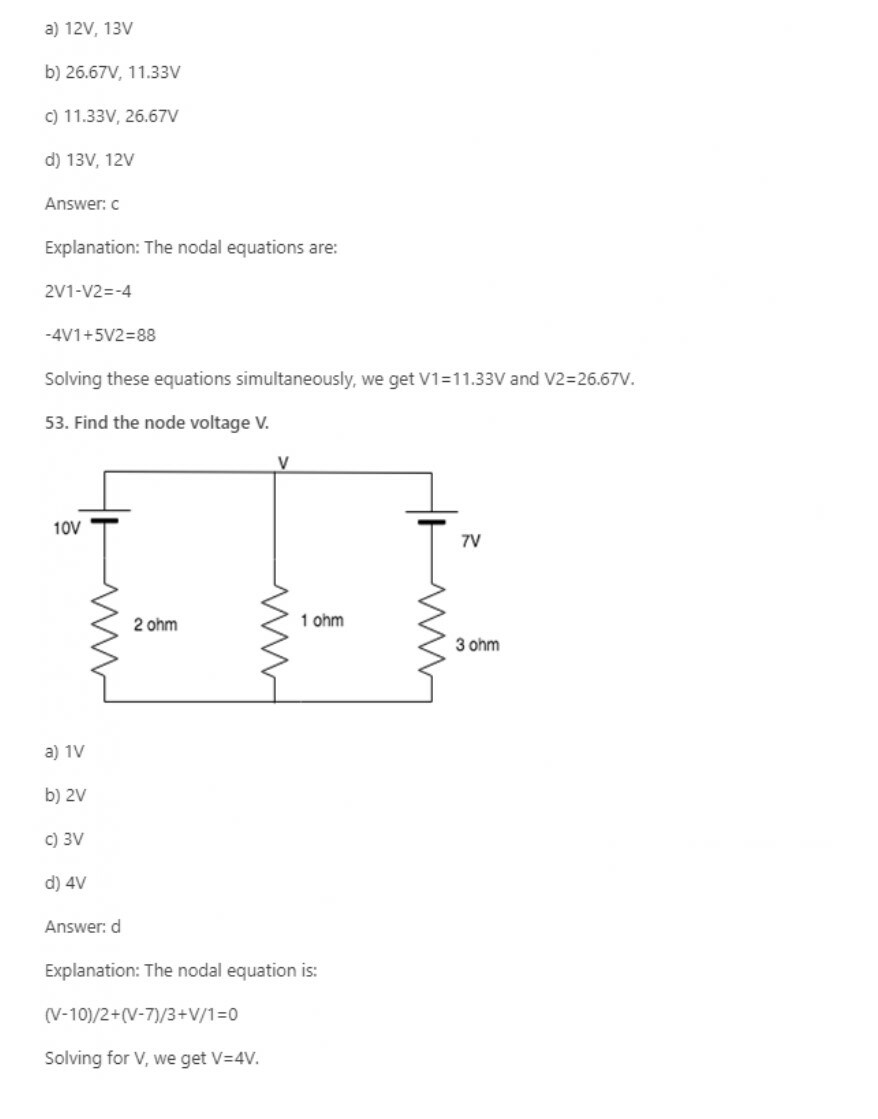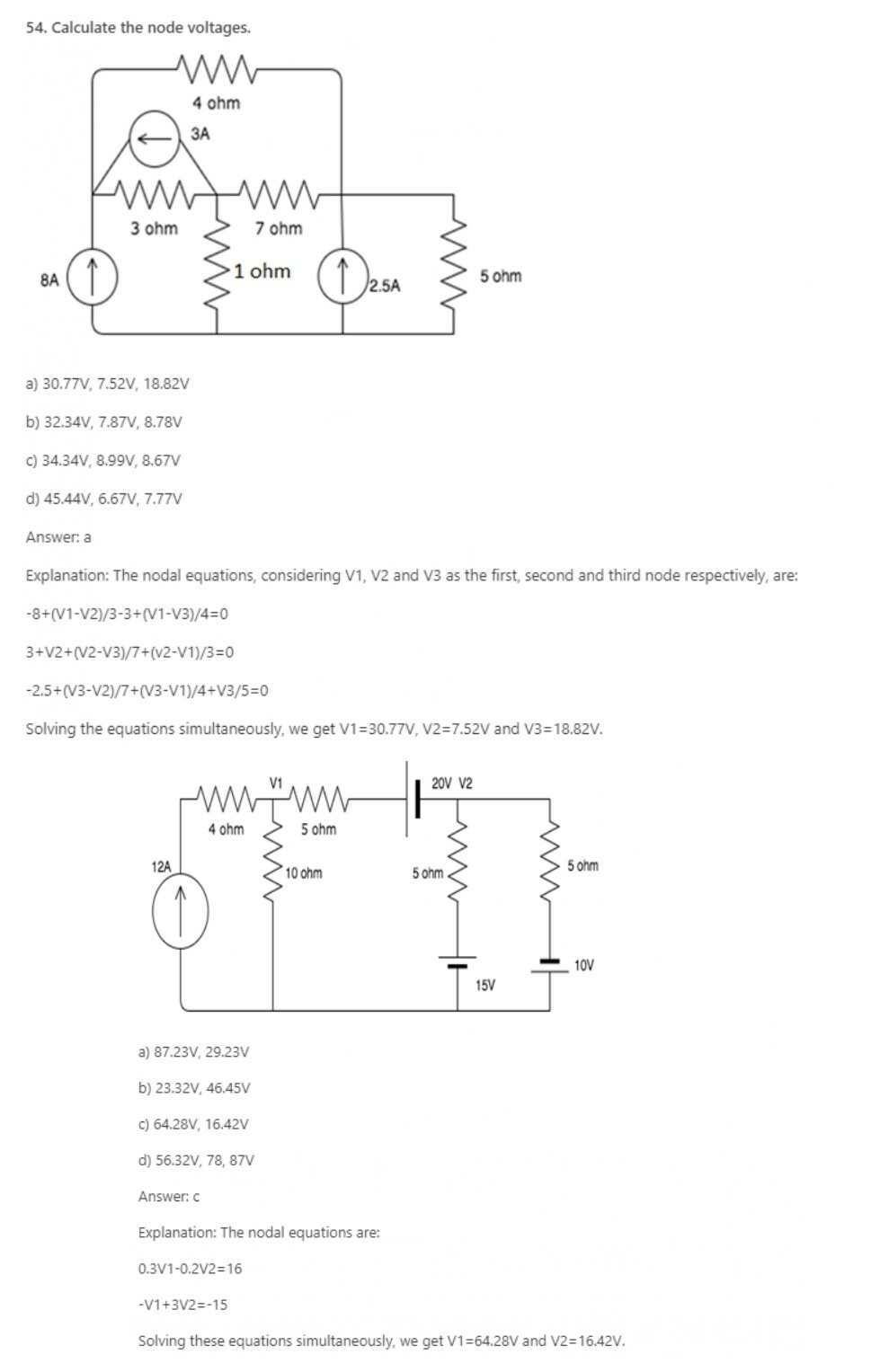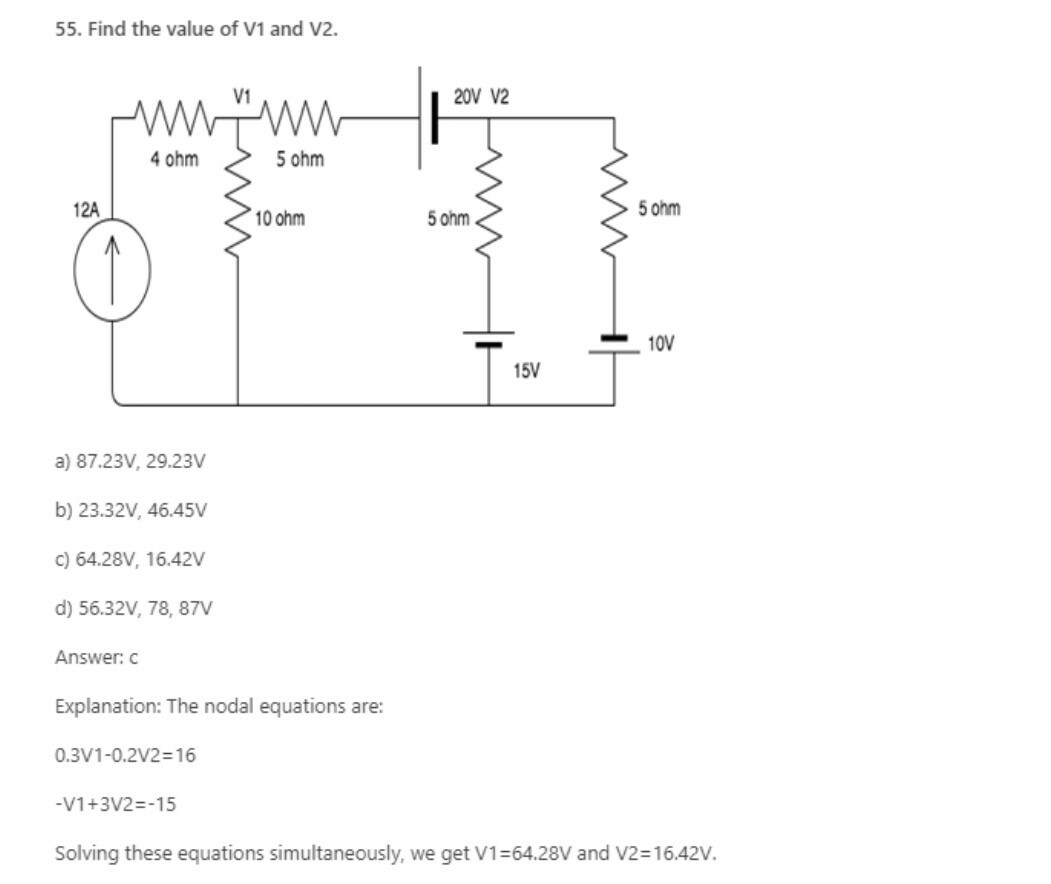56. Nodal analysis is generally used to determine_______

a) Voltage

b) Current

c) Resistance

d) Power

Explanation: Nodal analysis uses Kirchhoff’s Current Law to find all the node voltages. Hence it is a method used to determine the voltage.

Get Engineering Viva Question with Answers

57. If there are 10 nodes in a circuit, how many equations do we get?

a) 10

b) 9

c) 8

d) 7

Explanation: One node is taken as reference node so, the number of equations we get is always one less than the number of nodes in the circuit, hence for 10 nodes we get 9 equations.

58. Nodal analysis can be applied for________

a) Planar networks

b) Non-planar networks

c) Both planar and non-planar networks

d) Neither planar nor non-planar networks

Explanation: Nodal analysis can be applied for both planar and non-planar networks since each node, whether it is planar or non-planar, can be assigned a voltage.

59. How many nodes are taken as reference nodes in a nodal analysis?

a) 1

b) 2

c) 3

d) 4

Explanation: In the nodal analysis, one node is treated as the reference node and the voltage at that point is taken as 0.

Python Programming for Complete Beginners

60. A voltage source connected in series with a resistor can be converted to a?

a) Current source in series with a resistor

b) Current source in parallel with a resistor

c) Voltage source in parallel with a resistor

d) Cannot be modified

Explanation: A voltage source connected in series can be converted to a current source connected in parallel using the relation obtained from Ohm’s law, that is V=IR. This equation shows that a voltage source connected in series has the same impact as a current source connected in parallel.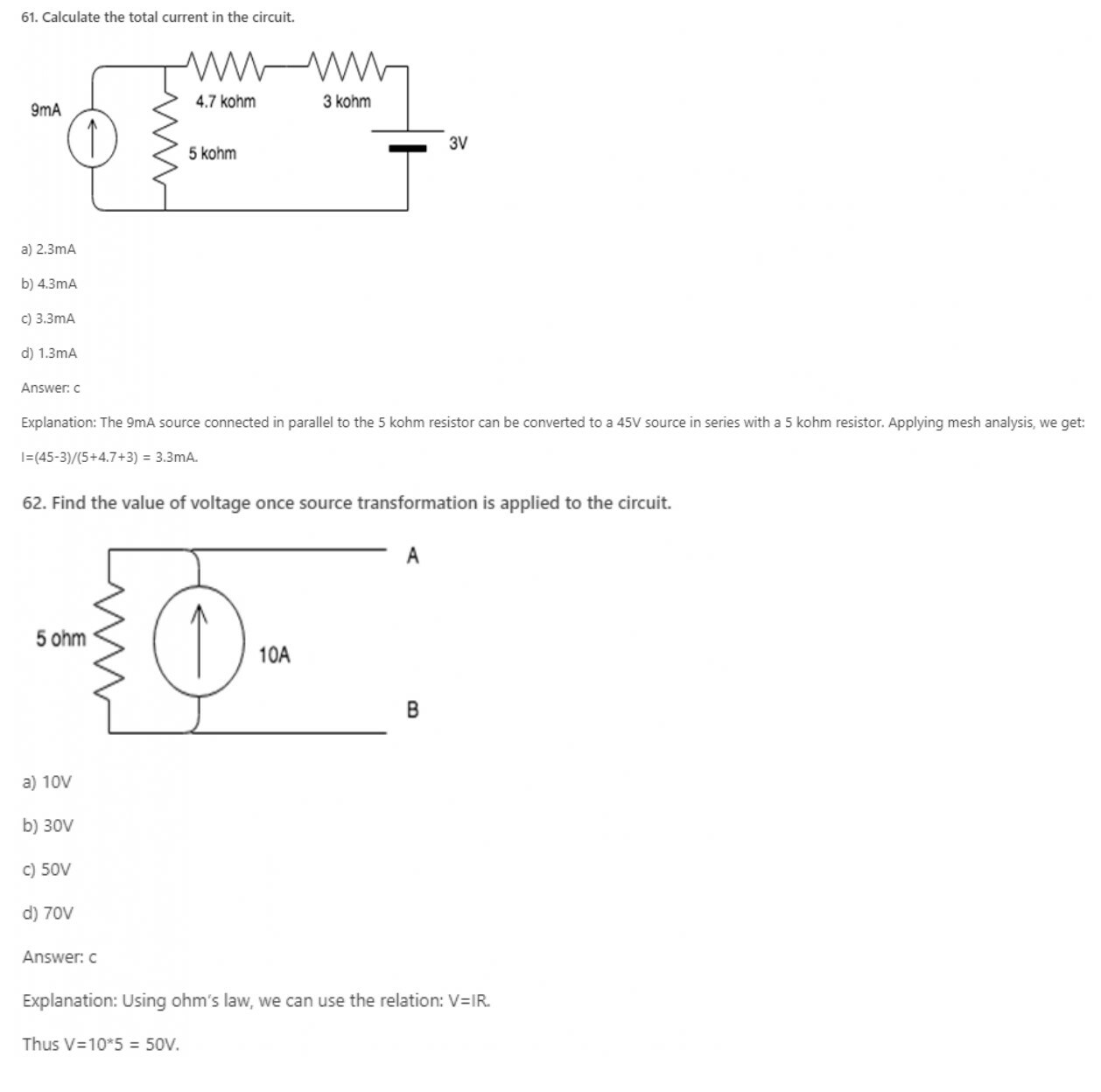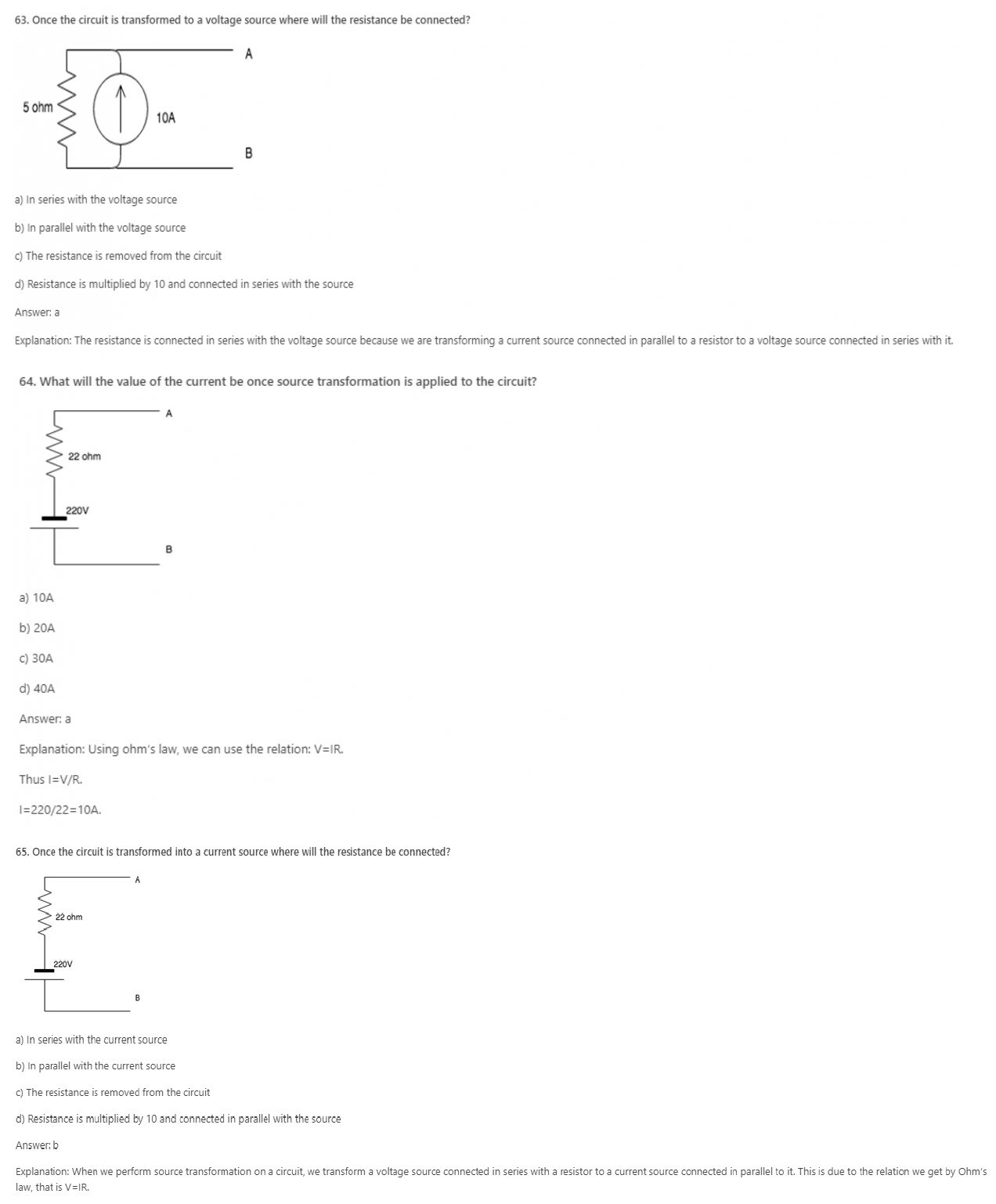66. A current source connected in parallel with a resistor can be converted to a?

a) Current source in series with a resistor

b) Voltage source in series with a resistor

c) Voltage source in parallel with a resistor

d) Cannot be modified

Explanation: A current source connected in parallel can be converted to a voltage source connected in series using the relation obtained from Ohm’s law, that is V=IR. This equation shows that a current source connected in parallel has the same impact as a voltage source connected in series.

67. A source transformation is_________

a) Unilateral

b) Bilateral

c) Unique

d) Cannot be determined

Explanation: A source transformation is bilateral because a voltage source can be converted to a current source and vice-versa.

68. In source transformation________

a) Voltage source remains the same

b) Current sources remain the same

c) Both voltage and current source remain the same

d) Resistances remain the same

Explanation: In source transformation, the value of the voltage and current sources change when changed from voltage to current source and current to voltage source but the value of the resistance remains the same.

69. If there are 3 10V sources connected in parallel then on source transformation__________

a) The effect of all the sources is considered

b) The effect of only one source is considered

c) The effect of none of the sources is considered

d) The effect of only 2 sources is considered.

Explanation: When voltages are connected in parallel, the effect of only one source is considered because the effect of the voltage remains the same when connected in parallel.

Python Programming for Complete Beginners

70.In superposition theorem, when we consider the effect of one voltage source, all the other voltage sources are ____________

a) Shorted

b) Opened

c) Removed

d) Undisturbed

Explanation: In superposition theorem when we consider the effect of one voltage source, all the other voltage sources are shorted and current sources are opened.

71.In superposition theorem, when we consider the effect of one current source, all the other voltage sources are ____________

a) Shorted

b) Opened

c) Removed

d) Undisturbed

72. In superposition theorem, when we consider the effect of one voltage source, all the other current sources are ____________

a) Shorted

b) Opened

c) Removed

d) Undisturbed

Explanation: In superposition theorem when we consider the effect of one voltage source, all the other current sources are opened and voltage sources are shorted.

73. In superposition theorem, when we consider the effect of one current source, all the other current sources are ____________

a) Shorted

b) Opened

c) Removed

d) Undisturbed

Explanation: In superposition theorem, whether we consider the effect of a voltage or current source, current sources are always opened and voltage sources are always shorted.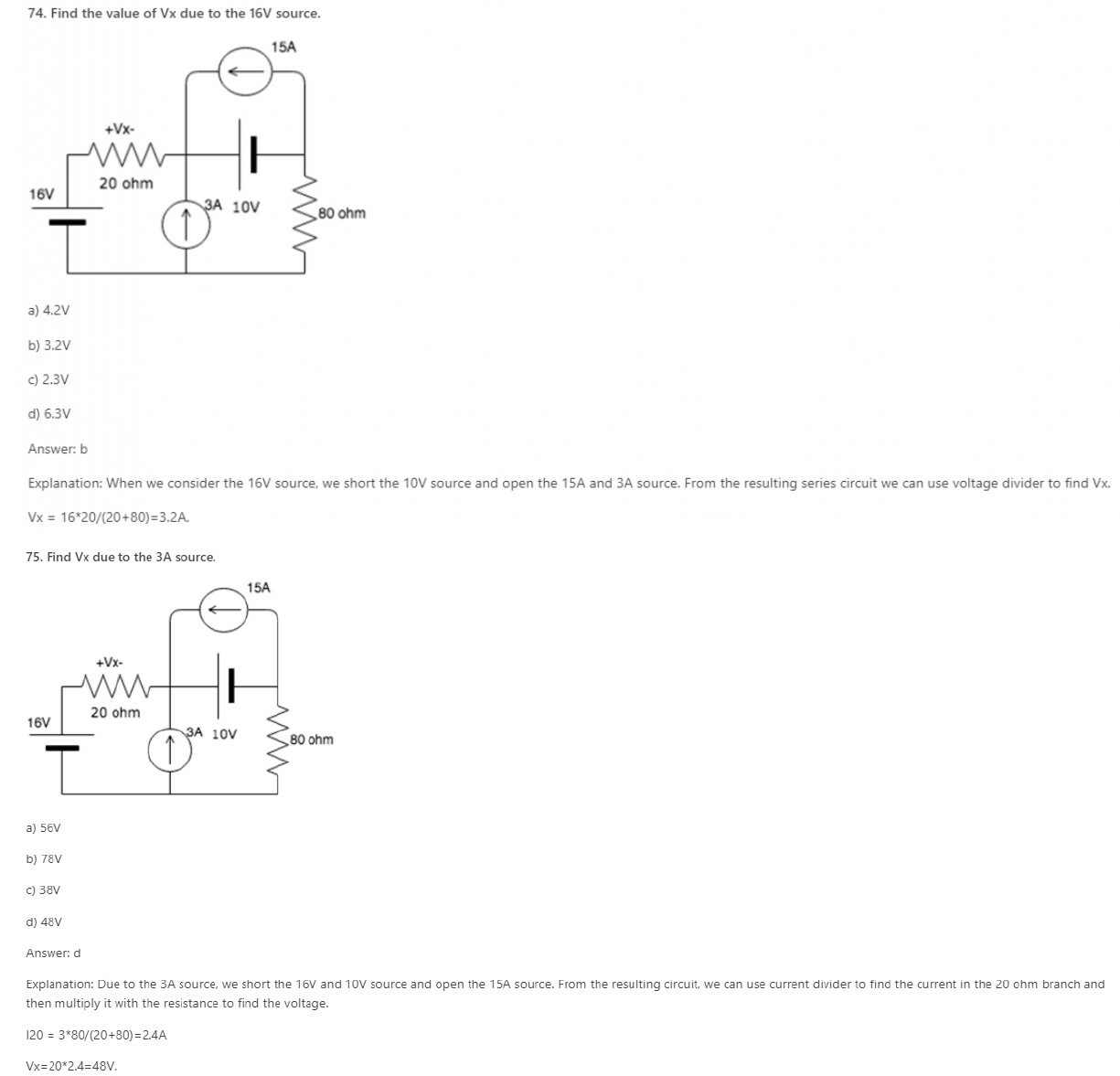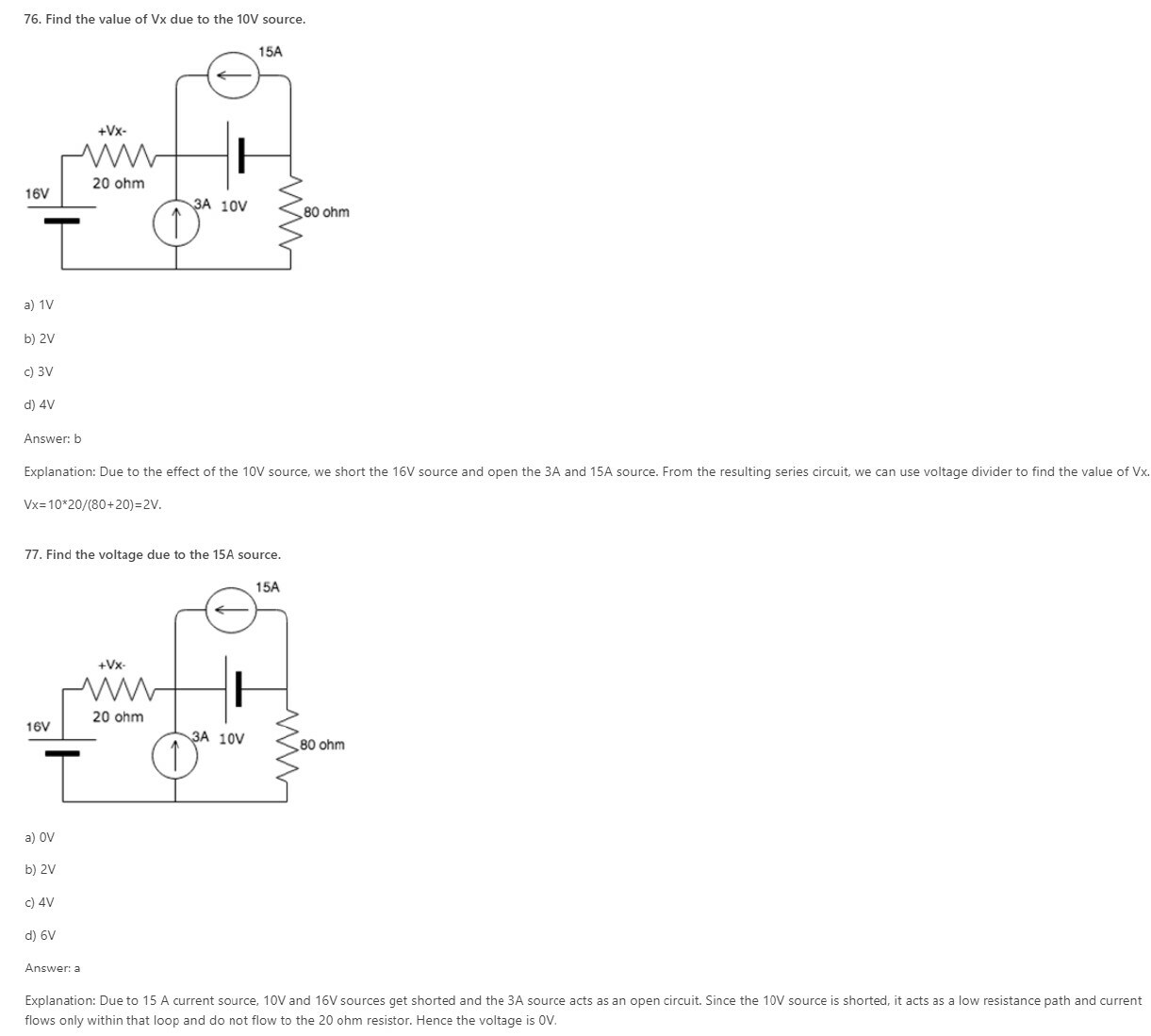78. Superposition theorem is valid for _________

a) Linear systems

b) Non-linear systems

c) Both linear and non-linear systems

d) Neither linear nor non-linear systems

Explanation: Superposition theorem is valid only for linear systems because the effect of a single source cannot be individually calculated in a non-linear system.

Python Programming for Complete Beginners

79. Superposition theorem does not work for ________

a) Current

b) Voltage

c) Power

d) Works for all: current, voltage and power

Explanation: Power across an element is not equal to the power across it due to all the other sources in the system. The power in an element is the product of the total voltage and the total current in that element.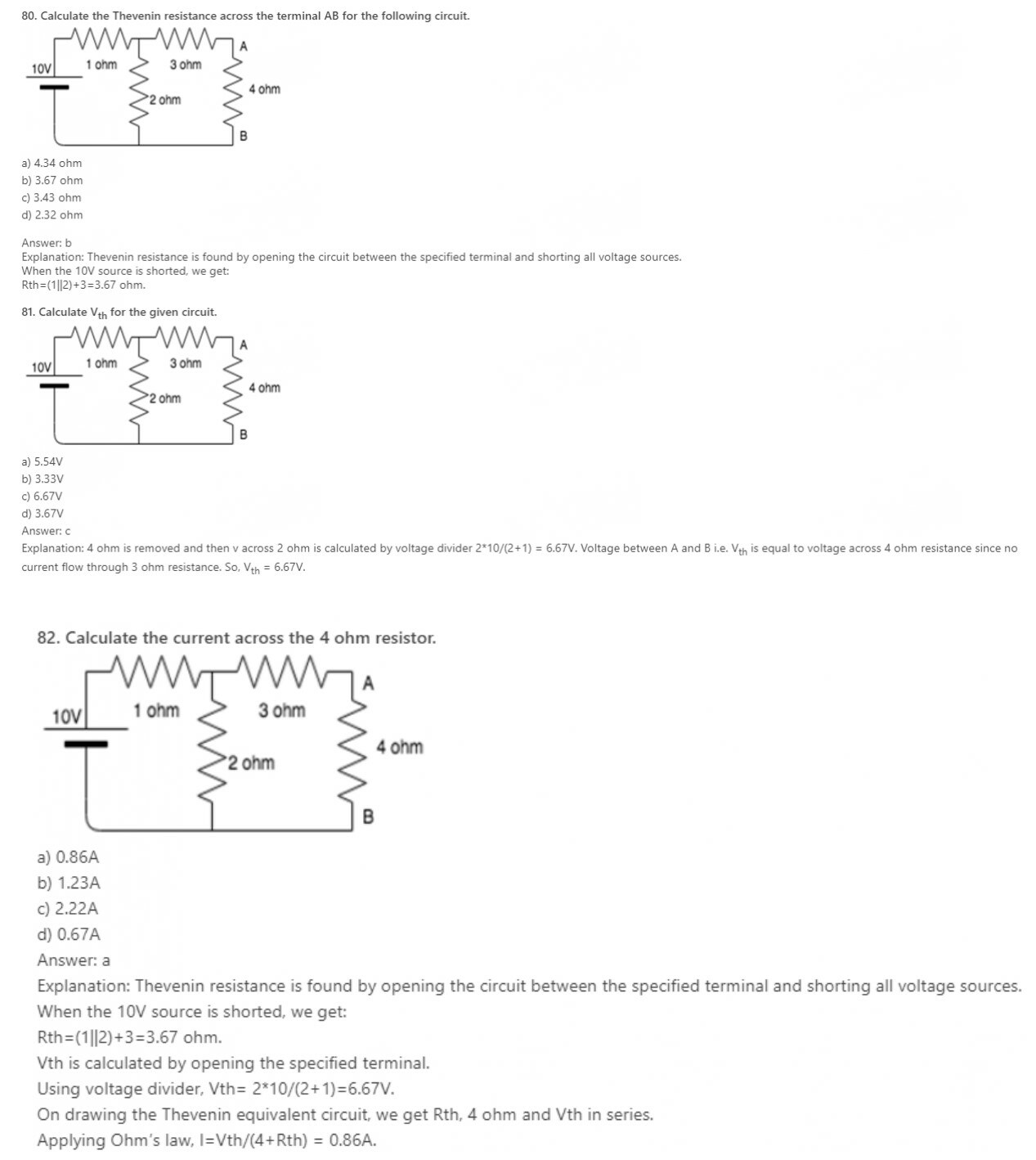83. The Thevenin voltage is the__________
a) Open circuit voltage
b) Short circuit voltage
c) Open circuit and short circuit voltage
d) Neither open circuit nor short circuit voltage
Explanation: Thevenin voltage is obtained by opening the specified terminals so it is open circuit voltage. It is not the short circuit voltage because if specified terminals are shorted voltage is equal to zero.

84. Thevenin resistance is found by ________
a) Shorting all voltage sources
b) Opening all current sources
c) Shorting all voltage sources and opening all current sources
d) Opening all voltage sources and shorting all current sources
Explanation: Ideal current sources have infinite internal resistance hence behave like an open circuit whereas ideal voltage sources have zero internal resistance hence behave as a short circuit.

85. Thevenin’s theorem is true for __________
a) Linear networks
b) Non-Linear networks
c) Both linear networks and nonlinear networks
d) Neither linear networks nor non-linear networks
Explanation: Thevenin’s theorem works for only linear circuit elements and not non-linear ones such as BJT, semiconductors etc.

86. In Thevenin’s theorem Vth is __________
a) Sum of two voltage sources
b) A single voltage source
c) Infinite voltage sources
d) 0
Explanation: Thevenin’s theorem states that a combination of voltage sources, current sources and resistors is equivalent to a single voltage source V and a single series resistor R.

87. Vth is found across the ____________ terminals of the network.
a) Input
b) Output
c) Neither input nor output
d) Either input or output
Explanation: According to Thevenin’s theorem, Vth is found across the output terminals of a network and not the input terminals.

Get Engineering Viva Question with Answers

88. Which of the following is also known as the dual of Thevenin’s theorem?
a) Norton’s theorem
b) Superposition theorem
c) Maximum power transfer theorem
d) Millman’s theorem
Explanation: Norton’s theorem is also known as the dual of Thevenin’s theorem because in Norton’s theorem we find short circuit current which is the dual of open circuit voltage-what we find in Thevenin’s theorem.

89. Can we use Thevenin’s theorem on a circuit containing a BJT?
a) Yes
b) No
c) Depends on the BJT
d) Insufficient data provided
Explanation: We can use Thevenin’s theorem only for linear networks. BJT is a non-linear network hence we cannot apply Thevenin’s theorem for it.

90. The Norton current is the_______
a) Short circuit current
b) Open circuit current
c) Open circuit and short circuit current
d) Neither open circuit nor short circuit current
Explanation: Norton current is obtained by shorting the specified terminals. So, it is the short circuit current. It is not the open circuit current because if specified terminals get open circuited then current is equal to zero.

Python Programming for Complete Beginners

91. Norton resistance is found by?
a) Shorting all voltage sources
b) Opening all current sources
c) Shorting all voltage sources and opening all current sources
d) Opening all voltage sources and shorting all current sources
Explanation: Ideal current sources have infinite internal resistance hence behave like an open circuit whereas ideal voltage sources have zero internal resistances hence behave as a short circuit. So, to obtain Norton resistance, all voltage sources are shorted and all current sources are opened.

92. Norton’s theorem is true for __________
a) Linear networks
b) Non-Linear networks
c) Both linear networks and nonlinear networks
d) Neither linear networks nor non-linear networks
Explanation: Norton’s theorem works for only linear circuit elements and not non-linear ones such as BJT, semiconductors etc.

93. In Norton’s theorem Isc is__________
a) Sum of two current sources
b) A single current source
c) Infinite current sources
d) 0
Explanation: Norton’s theorem states that a combination of voltage sources, current sources and resistors is equivalent to a single current source IN and a single parallel resistor RN.

94. Isc is found across the ____________ terminals of the network.
a) Input
b) Output
c) Neither input nor output
d) Either input or output
Explanation: According to Norton’s theorem, Isc is found through the output terminals of a network and not the input terminals.

95. Can we use Norton’s theorem on a circuit containing a BJT?
a) Yes
b) No
c) Depends on the BJT
d) Insufficient data provided
Explanation: We can use Norton’s theorem only for linear networks. BJT is a non-linear network hence we cannot apply Norton’s theorem for it.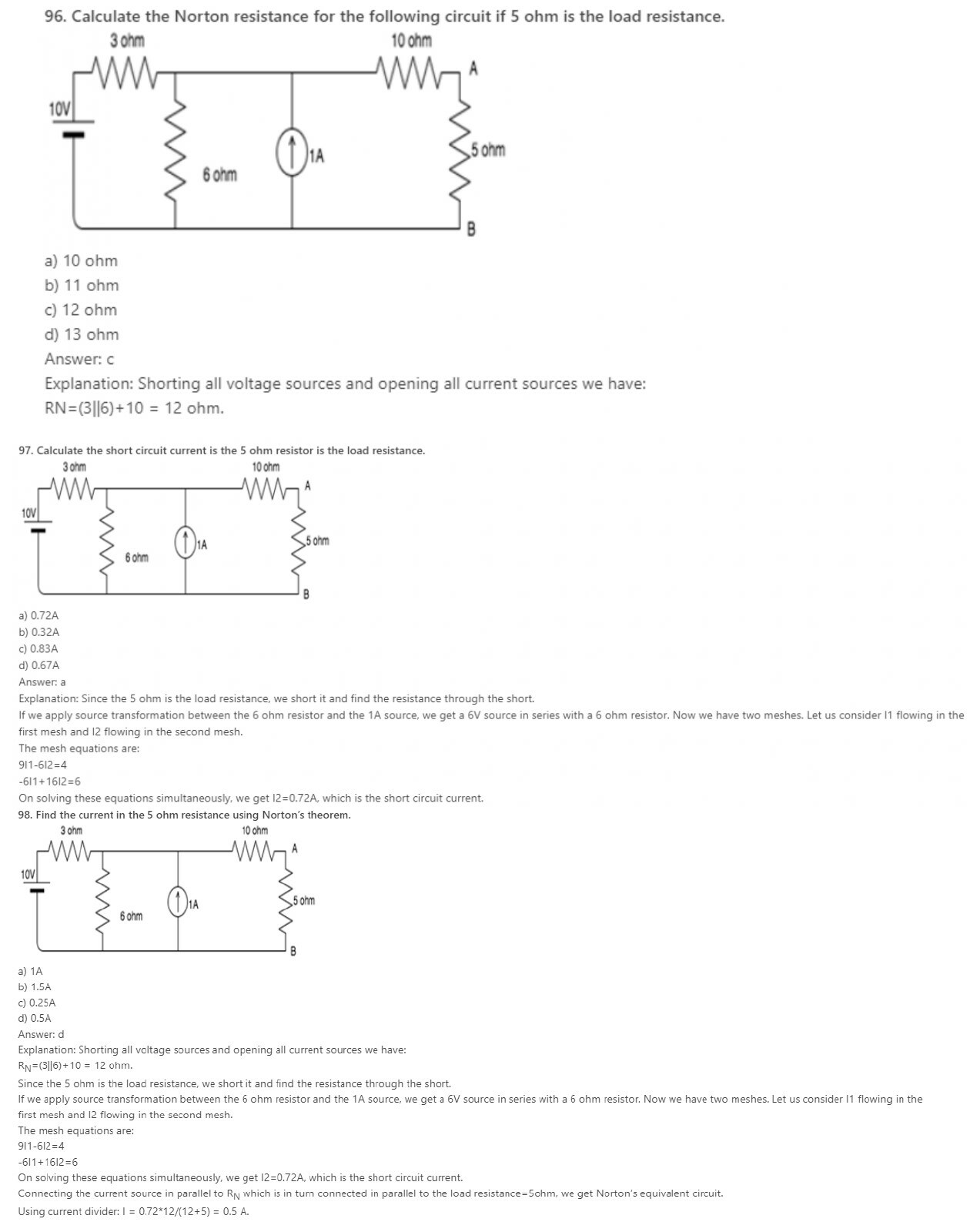99. Which of the following is also known as the dual of Norton’s theorem?
a) Thevenin’s theorem
b) Superposition theorem
c) Maximum power transfer theorem
d) Millman’s theorem
Explanation: Thevenin’s theorem is also known as the dual of Norton’s theorem because in Norton’s theorem we find short circuit current which is the dual of open circuit voltage-what we find in Thevenin’s theorem.

100. The maximum power drawn from source depends on __________
a) Value of source resistance
c) Both source and load resistance
d) Neither source or load resistance
Explanation: The maximum power transferred is equal to E2/4*RL. So, we can say maximum power depends on load resistance.

Python Programming for Complete Beginners

101. The maximum power is delivered to a circuit when source resistance is __________ load resistance.
a) Greater than
b) Equal to
c) Less than
d) Greater than or equal to
Explanation: The circuit can draw maximum power only when source resistance is equal to the load resistance.

102. If source impedance is a complex number Z, then load impedance is equal to _________
a) Z’
b) -Z
c) -Z’
d) Z
Explanation: When Source impedance is equal to Z, its load impedance is the complex conjugate of Z which is Z’. Only under this condition, maximum power can be drawn from the circuit.

103. If ZL=Zs’, then RL=?
a) -RL
b) Rs
c) -Rs
d) 0
Explanation: Rs is the real part of the complex number ZL. Hence when we find the complex conjugate the real part remains the same whereas the complex part acquires a negative sign.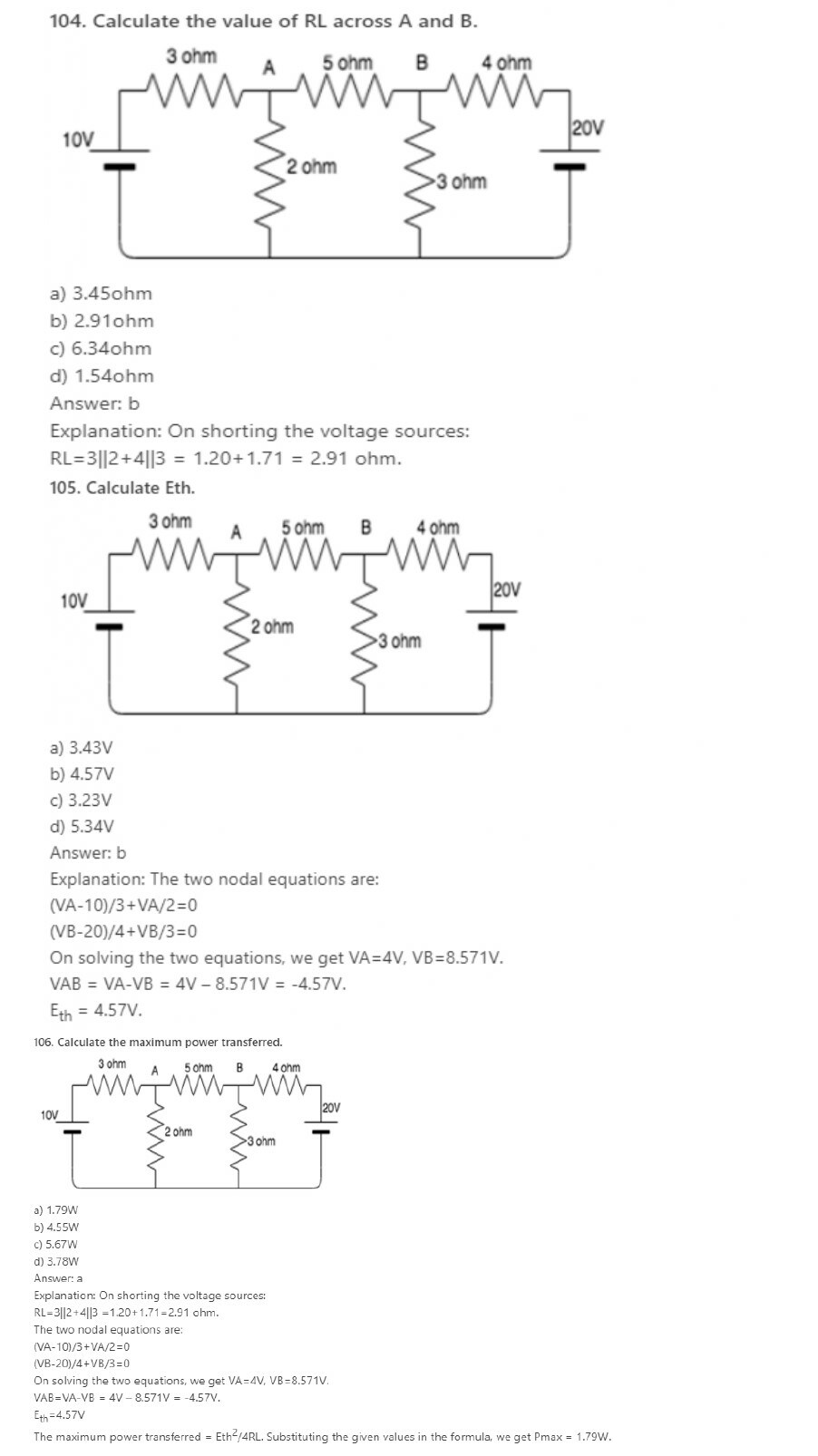107. Does maximum power transfer imply maximum efficiency?
a) Yes
b) No
c) Sometimes
d) Cannot be determined
Explanation: Maximum power transfer does not imply maximum efficiency. If the load resistance is smaller than source resistance, the power dissipated at the load is reduced while most of the power is dissipated at the source then the efficiency becomes lower.

108. Under the condition of maximum power efficiency is?
a) 100%
b) 0%
c) 30%
d) 50%
Explanation: Efficiency=(Power output/ Power input)*100.
Power Output=I2RL, Power Input=I2(RL+RS)
Under maximum power transfer conditions, RL=RS
Power Output=I2RL; Power Input=2*I2RL
Thus efficiency=50%.

109. Name some devices where maximum power has to be transferred to the load rather than maximum efficiency.
a) Amplifiers
b) Communication circuits
c) Both amplifiers and communication circuits
d) Neither amplifiers nor communication circuits
Explanation: Maximum power transfer to the load is preferred over maximum efficiency in both amplifiers and communication circuits since in both these cases the output voltage is more than the input.

Get Engineering Viva Question with Answers

#### AC Circuits

1. Find the average value of current when the current that are equidistant are 4A, 5A and 6A.
a) 5A
b) 6A
c) 15A
d) 10A
Explanation: The average value of current is the sum of all the currents divided by the number of currents. Therefore average current = (5+4+6)/3=5A.

2. What is the current found by finding the current in an equidistant region and dividing by n?
a) RMS current
b) Average current
c) Instantaneous current
d) Total current
Explanation: The average value of the current is the sum of all the currents divided by the number of currents.

3. RMS stands for ________
a) Root Mean Square
b) Root Mean Sum
c) Root Maximum sum
d) Root Minimum Sum
Explanation: RMS stands for Root Mean Square. This value of current is obtained by squaring all the current values, finding the average and then finding the square root.

4. What is the type of current obtained by finding the square of the currents and then finding their average and then fining the square root?
a) RMS current
b) Average current
c) Instantaneous current
d) Total current
Explanation: RMS stands for Root Mean Square. This value of current is obtained by squaring all the current values, finding the average and then finding the square root.

5. __________ current is found by dividing the area enclosed by the half cycle by the length of the base of the half cycle.
a) RMS current
b) Average current
c) Instantaneous current
d) Total current
Explanation: The average value of current is the sum of all the currents divided by the number of currents. Hence it can also be found by dividing the area enclosed by the half cycle by the length of the base of the half cycle.

6. What is the effective value of current?
a) RMS current
b) Average current
c) Instantaneous current
d) Total current
Explanation: RMS current is also known as the effective current. RMS stands for Root Mean Square. This value of current is obtained by squaring all the current values, finding the average and then finding the square root.

7. In a sinusoidal wave, average current is always _______ rms current.
a) Greater than
b) Less than
c) Equal to
d) Not related
Explanation: The average value of current is the sum of all the currents divided by the number of currents whereas RMS current is obtained by squaring all the current values, finding the average and then finding the square root. Hence RMS current is greater than average current.

8. For a rectangular wave, average current is ______ rms current.
a) Greater than
b) Less than
c) Equal to
d) Not relate

Explanation: The rms value is always greater than the average except for a rectangular wave, in which the heating effect remains constant so that the average and the rms values are the same.

9. Peak value divided by the rms value gives us?
a) Peak factor
b) Crest factor
c) Both peak and crest factor
d) Neither peak nor crest factor
Explanation: Peak and crest factor both mean the same thing. Hence the peak value divided by the rms value gives us the peak or crest factor.

10. Calculate the crest factor if the peak value of current is 10A and the rms value is 2A.
a) 5
b) 10
c) 5A
d) 10A
Explanation: We know that:
Crest factor = Peak value/RMS value.
Substituting the values from the given question, we get crest factor=5.

Python Programming for Complete Beginners

11. If maximum value of current is 5√2 A, what will be the value of RMS current?
a) 10 A
b) 5 A
c) 15 A
d) 25 A
Explanation: We know, value of RMS current =value of max current/√2
Substituting the value of max current we get, rms current = 5A.

12. If Im is the maximum value of a sinusoidal voltage, what is the instantaneous value?
a) i=Im/2
b) i=Imsinθ
c) i=Imcosθ
d) i=Imsinθ or i=Imcosθ
Explanation: The instantaneous value of a sinusoidal varying current is i=Imsinθ or i=Imcosθ where Im is the maximum value of current.

13. Average value of current over a half cycle is?
a) 0.67Im
b) 0.33Im
c) 6.7Im
d) 3.3Im
Explanation: Average current = ∫0πidθ/π = ∫0πImsinθ dθ/π = 2Im/π =0.67 Im.

14. What is the correct expression for the rms value of current?
a) Irms=Im/2
b) Irms=Im/√2
c) Irms=Im/4
d) Irms=Im
Explanation: Irms2 = ∫0πdθ i2/2π = Im2/2
Irms=Im/√2.

15. Average value of current over a full cycle is?
a) 0.67Im
b) 0
c) 6.7Im
d) 3.3Im
Explanation: Average of sine or cosine over a period is zero so, average value of current over full cycle is zero.

16. What is the correct expression for the form factor?
a) Irms * Iav
b) Irms / Iav
c) Irms + Iav
d) Irms – Iav
Explanation: The correct expression for form factor is Irms/Iav where Irms is the rms value of the current and Iav is the average current.

17. For a direct current, the rms current is ________ the mean current.
a) Greater than
b) Less than
c) Equal to
d) Not related to
Explanation: For a direct current, the mean current value is the same as that of the rms current.

Get Engineering Viva Question with Answers

18. For a direct current, the rms voltage is ________ the mean voltage.
a) Greater than
b) Less than
c) Equal to
d) Not related to
Explanation: For a direct current, the mean voltage value is the same as that of the rms voltage.

19. What is the value of the form factor for sinusoidal current?
a) π/2
b) π/4
c) 2π
d) π/√2
Explanation: For sinusoidal current, Irms=Im/√2
Iav=√2 Im/π
So, form factor = Irms/Iav = π/2.

20. If the maximum value of the current is 5√2 A, what will be the value of the average current?
a) 10/π A
b) 5/π A
c) 15/π A
d) 25/π A
Explanation: We know, the value of the average current = value of max current *√2 /π
Substituting the value of max current we get, rms current = 10/π A.

Python Programming for Complete Beginners

21. What is the resonance frequency of ac circuit?
a) 1/√LC
b) √(L/C)
c) √LC
d) LC
Explanation: At resonance, XL=XC
ωL=1/ωC
ω=1/√LC.

22. What is impedance at resonance?
a) maximum
b) minimum
c) zero
d) cannot be determined
Explanation: At resonance, XL=XC
Z2=R2+(XL-XC)2
Z=R So Z is minimum at resonance.

23. What is the value of impedance at resonance?
a) XL
b) XC
c) R
d) 0
Explanation: At resonance, XL=XC
Z2=R2+(XL-XC)2
Z=R So Z is minimum at resonance.

24. What is φ in terms of voltage?
a) φ=cos-1V/VR
b) φ=cos-1V*VR
c) φ=cos-1VR/V
d) φ=tan-1V/VR
Explanation: Form the voltage triangle, we get cosφ= VR/V.
Hence φ=cos-1VR/V.

25. What is tanϕ for RC circuit?
a) XC/R
b) XL/R
c) R/Z
d) Z/R
Explanation: From the impedance triangle, height gives capacitive reactance and base gives resistance.
tanϕ=XC/R.

26. What is the resonance condition?
a) When XL>XC
b) When XL<XC
c) When XL=XC
d) When XC=infinity
Explanation: The current is in phase with the voltage when the capacitive reactance is in equal to the inductive reactance. This is known as resonance condition.

27. What is the frequency in resonance condition?
a) Minimum
b) Maximum
c) Cannot be determined
d) Zero
Explanation: At resonance condition, the frequency is maximum since the inductive reactance is equal to the capacitive reactance. XL=XC.

28. A resistance of 7 ohm is connected in series with an inductance of 31.8mH. The circuit is connected to a 100V 50Hz sinusoidal supply. Calculate the current in the circuit.
a) 2.2A
b) 4.2A
c) 6.2A
d) 8.2A
Explanation: XL=2*π*f*L = 10 ohm. Z2=(R2+XL2)
Therefore the total impedance Z = 12.2ohm.
V=IZ, therefore I=V/Z=100/12.2 = 8.2A.

29. A resistance of 7 ohm is connected in series with an inductance of 31.8mH. The circuit is connected to a 100V 50Hz sinusoidal supply. Calculate the phase difference.
a) -55.1
b) 55.1
c) 66.1
d) -66.1
Explanation: φ=tan-1(XL/R)=55.1
Since this is an inductive circuit, the current will lag, hence φ= -55.1.

30. A resistance of 7 ohm is connected in series with an inductance of 31.8mH. The circuit is connected to a 100V 50Hz sinusoidal supply. Calculate the voltage across the resistor.
a) 31.8V
b) 57.4V
c) 67.3V
d) 78.2V
Explanation: XL=2*π*f*L = 10 ohm. Z2=(R2+XL2)
Therefore, the total impedance Z = 12.2ohm.
V=IZ, therefore I=V/Z=100/12.2 = 8.2A. Voltage across resistor = 8.2*7 = 57.4V.

Python Programming for Complete Beginners

31. A resistance of 7 ohm is connected in series with an inductance of 31.8mH. The circuit is connected to a 100V 50Hz sinusoidal supply. Calculate the voltage across the inductor.
a) 52V
b) 82V
c) 65V
d) 76V
Explanation: XL=2*π*f*L = 10 ohm. Z2=(R2+XL2)
Therefore, the total impedance Z = 12.2ohm.
V=IZ, therefore I=V/Z=100/12.2 = 8.2A. Voltage across inductor = 8.2*10 = 82V.

32. A resistance of 7 ohm is connected in series with an inductance of 31.8mH. The circuit is connected to a x V 50Hz sinusoidal supply. The current in the circuit is 8.2A. Calculate the value of x.
a) 10V
b) 50V
c) 100V
d) 120V
Explanation: XL=2*π*f*L= 10 ohm. Z2=(R2+XL2)
Therefore, the total impedance Z = 12.2ohm.
V=IZ, therefore V = 12.2*8.2 = 100V.

33. Which, among the following, is the correct expression for φ.
a) φ=tan-1 (XL/R)
b) φ=tan-1 (R/XL)
c) φ=tan-1 (XL*R)
d) φ=cos-1 (XL/R)
Explanation: From the impedance triangle, we get tanφ= XL/R.
Hence φ=tan-1 (XL/R).

34. For an RL circuit, the phase angle is always ________
a) Positive
b) Negative
c) 0
d) 90
Explanation: For a series resistance and inductance circuit the phase angle is always a negative value because the current will always lag the voltage.

35. What is φ in terms of voltage?
a) φ=cos-1V/VR
b) φ=cos-1V*VR
c) φ=cos-1VR/V
d) φ=tan-1V/VR
Explanation: From the voltage triangle, we get cosφ= VR/V.
Hence φ=cos-1VR/V.

36. What is sinϕ from impedance triangle?
a) XL/R
b) XL/Z
c) R/Z
d) Z/R
Explanation: In the Impedance triangle, Base is R, Hypotenuse is Z, Height is XL.
So, sinϕ = XL/Z.

Get Engineering Viva Question with Answers

#### Star and Delta

1. In a balanced three-phase system-delta load, if we assume the line voltage is VRY = V∠0⁰ as a reference phasor. Then the source voltage VYB is?
a) V∠0⁰
b) V∠-120⁰
c) V∠120⁰
d) V∠240⁰

Explanation: As the line voltage VRY = V∠0⁰ is taken as a reference phasor. Then the source voltage VYB is V∠-120⁰.

2. In the question 1, the source voltage VBR is?
a) V∠120⁰
b) V∠240⁰
c) V∠-240⁰
d) V∠-120⁰

Explanation: As the line voltage VRY = V∠0⁰ is taken as a reference phasor. Then the source voltage VBR is V∠-240⁰.

3. In a delta-connected load, the relation between line voltage and the phase voltage is?
a) line voltage > phase voltage
b) line voltage < phase voltage
c) line voltage = phase voltage
d) line voltage >= phase voltage

Explanation: In a delta-connected load, the relation between line voltage and the phase voltage is line voltage = phase voltage.

4. If the load impedance is Z∠Ø, the current (IR ) is?
a) (V/Z)∠-Ø
b) (V/Z)∠Ø
c) (V/Z)∠90-Ø
d) (V/Z)∠-90+Ø

Explanation: As the load impedance is Z∠Ø, the current flows in the three load impedances and the current flowing in the R impedance is IR = VBR∠0⁰/Z∠Ø = (V/Z)∠-Ø.

5. In the question 4, the expression obtained for current (IY) is?
a) (V/Z)∠-120+Ø
b) (V/Z)∠120-Ø
c) (V/Z)∠120+Ø
d) (V/Z)∠-120-Ø

Explanation: As the load impedance is Z∠Ø, the current flows in the three load impedances and the current flowing in the Y impedance is IY = VYB∠120⁰/Z∠Ø = (V/Z)∠-120-Ø.

6. In the question 4, the expression obtained for current (IB) is?
a) (V/Z)∠-240+Ø
b) (V/Z)∠-240-Ø
c) (V/Z)∠240-Ø
d) (V/Z)∠240+Ø

Explanation: As the load impedance is Z∠Ø, the current flows in the three load impedances and the current flowing in the B impedance is IB = VBR∠240⁰/Z∠Ø = (V/Z)∠-240-Ø.

7. A three phase, balanced delta connected load of (4+j8) Ω is connected across a 400V, 3 – Ø balanced supply. Determine the phase current IR . Assume the phase sequence to be RYB.
a) 44.74∠-63.4⁰A
b) 44.74∠63.4⁰A
c) 45.74∠-63.4⁰A
d) 45.74∠63.4⁰A

Explanation: Taking the line voltage VRY = V∠0⁰ as a reference VRY = 400∠0⁰V, VYB = 400∠-120⁰V and VBR = 400∠-240⁰V. Impedance per phase = (4+j8) Ω = 8.94∠63.4⁰Ω. Phase current IR = (400∠0o)/(8.94∠63.4o )= 44.74∠-63.4⁰A.

8. In the question 7, determine the phase current IY.
a) 44.74∠183.4⁰A
b) 45.74∠183.4⁰A
c) 44.74∠183.4⁰A
d) 45.74∠-183.4⁰A

Explanation: Taking the line voltage VRY = V∠0⁰ as a reference VRY = 400∠0⁰V, VYB = 400∠-120⁰V and VBR = 400∠-240⁰V. Impedance per phase = (4+j8)Ω = 8.94∠63.4⁰Ω. Phase current IY = (400∠120o)/(8.94∠63.4o )= 44.74∠-183.4⁰A.

9. In the question 7, determine the phase current IB.
a) 44.74∠303.4⁰A
b) 44.74∠-303.4⁰A
c) 45.74∠303.4⁰A
d) 45.74∠-303.4⁰A

Explanation: Taking the line voltage VRY = V∠0⁰ as a reference VRY = 400∠0⁰V, VYB = 400∠-120⁰V and VBR = 400∠-240⁰V. Impedance per phase = (4+j8) Ω = 8.94∠63.4⁰Ω. Phase current IB = (400∠240o)/(8.94∠63.4o)= 44.74∠-303.4⁰A.

10. Determine the power (kW) drawn by the load.
a) 21
b) 22
c) 23
d) 24

Explanation: Power is defined as the product of voltage and current. So the power drawn by the load is P = 3VPhIPhcosØ = 24kW.
Python Programming for Complete Beginners

11. For a good 0.5 micro-farad paper capacitor, the ohm-meter reading should

a) Go quickly to 100 ohms and remain there
b) Show low resistance momentarily and back off to a very high resistance
c) Not move at all
d) Show very high resistance first and then low

12. Which of the following error may arise in wattmeter if it is not compensated for the errors?

a) Voltage coil inductance
b) Voltage coil capacitance
c) Eddy currents
d) (a), (b) and (c)

13. Which of the following is methods is the commonest method of measuring three balanced or unbalanced power?

a) One wattmeter method
b) Two wattmeter method
c) Three wattmeter method
d) Ammeter method

14. One-Wattmeter method is used to measure

a) The power when load is balance in three phase circuit
b) The power when load is unbalanced in three phase circuit
c) (a) or (b)
d) Single phase power with balanced load

15. The reactive power can be measured with wattmeter when voltage across voltage coil is adjusted to be out of phase with the current by

a) 90°
b) 180°
c) 45°
d) 0°
e) 120°

16.The change of frequency affects the circuit parameters of wattmeter connected to measure power of three-phase AC system

a) True
b) False

17. Which of the following devices are required to measure three phase balance power?

a) One wattmeter
b) One wattmeter and one voltage transformer of 1:1 ratio
c) One wattmeter and two current transformers of 1:1 ratio
d) Either (b) or (c)

18. The total power P measured in Y star three-phase circuit is given by

a) P = 3VI cos ⁡ϕ
b) P = √I cos ⁡ϕ
c) P = √3VI cos ⁡ϕ
d) P = √2VI cos⁡ ϕ

Where V and I are the line voltage and line current respectively.

19.In the above question, the power in delta three-phase circuit is given by

a) P = 3 VI cos ⁡ϕ
b) P = √3 VI cos ⁡ϕ
c) P = √2 VI cos ⁡ϕ
d) P = √3 VI sin ⁡ϕ

20. The dynamometer wattmeter can be used to measure

a) AC power only
b) DC power only
c) AC or DC power
d) AC power of single-phase circuits

Get Engineering Viva Question with Answers

#### Transformers

1. How many design principles are present in the current transformers?
a) 2
b) 3
c) 4
d) 5

Explanation: There are 5 design principles present in the current transformers. They are core design, secondary current rating, primary current rating, windings and behavior of the transformer under short circuit current.

2. What should be done in order to reduce the errors in the core?
a) armature mmf is to kept low
b) field mmf to be kept high
c) the exciting mmf is to be kept low
d) the field mmf is to be kept high

Explanation: The errors in the core are reduced by keeping the exciting mmf low. This can take place with the core having a low reluctance and low iron loss.

3. How many classifications are the magnetic alloys used in the current transformers classified into?
a) 3
b) 2
c) 4
d) 5

Explanation: The magnetic alloys used in the current transformers are divided into 3 types. They are hot rolled silicon steel, cold rolled grain oriented silicon steel and nickel iron alloys.

4. What is the material used in the transformer when the transformer errors should be small?
a) mumetal cores
b) steel cores
c) permender cores
d) presshamn cores

Explanation: The mumetal cores are commonly used when it is essential that transformer errors shall be small. Mumetal has the properties of high permeability, low loss and small retentivity.

5. What is the relation of the secondary winding leakage reactance and secondary circuit impedance?
a) secondary winding leakage reactance is directly proportional to the secondary circuit impedance
b) secondary winding leakage reactance is indirectly proportional to the secondary circuit impedance
c) secondary winding leakage reactance is directly proportional to the square of the secondary circuit impedance
d) secondary winding leakage reactance is indirectly proportional to the square of the secondary circuit impedance

Explanation: The secondary winding leakage reactance is directly proportional to the secondary circuit impedance. In secondary winding the leakage reactance is reduced and in turn the secondary circuit impedance is reduced.

6. The ring shaped cores are made use of in the reduction of the secondary winding leakage reactance and secondary impedance.
a) true
b) false

Explanation: The secondary winding leakage reactance is directly proportional to the secondary impedance. The ring shaped cores around which the toroidal secondary windings of one or more layers are uniformly distributed.

7. What type of core is employed when the performance standard required is not so high?
a) rectangular strips
b) c-shaped sections
c) rectangular strips or c-shaped sections
d) rectangular strips and c-shaped sections

Explanation: Ring core type is used only for the high performance operation. The rectangular strips or c-shaped sections are used when the standard of performance required is not so high.

8. What should the magnetic path be in order to reduce the core reluctance?
a) length of the magnetic path in core should be low
b) length of the magnetic path in core should be medium
c) length of the magnetic path in core should be high
d) length of the magnetic path in core should be very high

Explanation: The length of the magnetic path in core should be as small as possible. This reduces the core reluctance of the current transformer.

9. What is the value of the rated secondary current?
a) 1 A
b) 2 A
c) 3 A
d) 5 A

Explanation: The rating of the secondary current is the maximum current that can be passed through the secondary windings. It is 5 A for the current transformer.

10. What are the disadvantages of the low rated secondary current transformer?
a) high cost
b) high voltages
c) high voltages or high cost
d) high voltages and high cost

Explanation: When there is a low secondary current rating in the current transformers they produces high voltages if the secondary is left open. It is also costly to produce the windings because of the extra time involved in the making.
Python Programming for Complete Beginners

11. What is the ideal condition with respect to the primary current rating?
a) ratio of secondary mmf to primary mmf should be high
b) ratio of secondary mmf to primary mmf should be low
c) ratio of excitation mmf to primary mmf should be high
d) ratio of excitation mmf to primary mmf should be low

Explanation: The primary current rating depends on exciting mmf and primary mmf. The ratio of the exciting mmf to the primary mmf should be low.

12. What is the rating of the primary current in the current transformer?
a) 200 A
b) 300 A
c) 400 A
d) 500 A

Explanation: The rating of the primary current is minimum 500 A. If the rating is less than 500 A, then multiturn primary windings and rating is above than 500 A, then single turn winding is enough.

13. How many types are the current transformers classified into?
a) 2
b) 3
c) 4
d) 5

Explanation: The current transformers are classified into 2 types. They are wound type and bar type.

14. What is the wound type current transformer?
a) primary winding having one full turn wound on core
b) primary winding having more than one full turn wound on core
c) secondary winding having one full turn wound on core
d) secondary winding having more than one full turn wound on core

Explanation: The wound type current transformer is one of the classifications of the current transformers. The primary winding has more than one full turn wound on core.

15. What is the bar type current transformer?
a) primary winding consists of a rod of suitable size and material
b) primary winding consists of a bar of suitable size and material
c) secondary winding consists of a rod of suitable size and material
d) secondary winding consists of a bar of suitable size and material

Explanation: The bar type current transformer is one of the classifications of the current transformers. In bar type winding, primary winding consists of a bar of suitable size and material.

16. How many commonly used shapes of current transformer are present?
a) 1
b) 2
c) 3
d) 4

Explanation: The current transformers consist of 3 commonly used shapes. They are stadium, circular, rectangular orifices.

17. What material is made use of for the lamination in the current transformer?
a) cold rolled steels
b) hot rolled steels
c) copper
d) hot iron

Explanation: The current transformer consists of stacks of laminations. The lamination used in the current transformer is hot rolled steel.

18. What is the insulation material used in the current transformer?
a) elephantide
b) presspahn
c) elephantide and presspahn
d) elephantide or presspahn

Explanation: The insulation in current transformer is by means of end collars and circumferential wraps. The insulation material used in elephantide or presspahn.

19. What is the additional usage of the presspahn material used as insulation material?
a) lamination
b) to reduce the losses
c) to protect secondary winding conductor from mechanical damage
d) to protect secondary winding conductor from electrical damage

Explanation: The presspahn is used as insulating material in the current transformer. In addition to that the presspahn is also used to protect the secondary winding conductor from mechanical damage.
Python Programming for Complete Beginners

20. What is the other name of the ring type current transformer?
a) brush transformer
b) cloud transformer
c) circular transformer
d) bushing transformer

Explanation: The ring type current transformer is one type of current transformer. It is also known as the bushing transformer.

21. How many faces are present in the split core current transformer?
a) 2
b) 3
c) 4
d) 5

Explanation: The split core transformer consists of a split core. The split half consists of 2 finely grounded or lapped gap faces.

22. The current transformers are assembled on to the secondary conductors “on site” for either permanent or temporary duty.
a) true
b) false

Explanation: The current transformers are assembled on to the primary conductors. They are assembled “on site” for the permanent or temporary duty.

23. What is the insulation material on the primary conductor?
a) bakelized paper tube
b) resin
c) bakelized paper tube and resin
d) bakelized paper tube or resin

Explanation: The insulation material on the primary conductors is generally made up of the bakelized paper tube. It can also be made use of resin directly moulded on the bar.

24. How is the reluctance of the interleaved corner related with the magnetizing current?
a) reluctance of the interleaved corner is directly proportional to the magnetizing current
b) reluctance of the interleaved corner is indirectly proportional to the magnetizing current
c) reluctance of the interleaved corner is directly proportional to the square of the magnetizing current
d) reluctance of the interleaved corner is indirectly proportional to the square of the magnetizing current

Explanation: The reluctance of the interleaved corner is directly proportional to the magnetizing current. As the reluctance is being reduced it in turn reduces the magnetizing current.

25. To reduce the peak voltage between layers, the secondary winding is being sectionalized.
a) true
b) false

Explanation: The large number of secondary turns requiring more than 1 winding layer, the secondary winding is sectionalized. This is because to reduce the peak voltage between layers.
Get Engineering Viva Question with Answers

26. What is the use of the turns compensation in current transformer?
a) to increases the ratio error
b) to reduce the ratio error
c) to increase the phase angle error
d) to reduce the phase angle error

Explanation: There are 2 types of errors in the current transformer. The turns compensation is used to reduce the ratio error.

27. What is the formula of the actual ratio?
a) actual ratio = turns ratio + load current * secondary current
b) actual ratio = turns ratio * load current * secondary current
c) actual ratio = turns ratio + load current / secondary current
d) actual ratio = turns ratio / load current * secondary current

Explanation: The turns ratio is first calculated. Next the load current and secondary current is calculated and on substitution gives the actual ratio.

28. What happens if the number of secondary turns is reduced?
a) the primary turns is reduced
b) the output is reduced
c) the efficiency is reduced
d) the transformation ratio is reduced

Explanation: The reduction of the number of secondary turns reduces the transformation ratio. If the number of secondary turns reduces by 1 percent the actual transformation ratio reduces by equal percentage.

29. What is the best number of secondary turns of the current transformer?
a) 1
b) 2
c) 1 or 2 less than the number such that the turns ratio is equal to the nominal current ratio
d) 1 or 2 more than the number such that the turns ratio is equal to the nominal current ratio

Explanation: The best number of secondary turns of the current transformer is 1 or 2 less than the number such that the turns ratio is equal to the nominal current ratio. For example in a 1000/5 current transformer, the secondary turns number would be 198 or 199 rather than 200.

30. The phase angle error is significantly affected by the small change in secondary turns.
a) true
b) false

Explanation: There are 2 types of errors which is the ratio error and phase angle error. The phase angle error is not significantly effected by a small change in secondary turns.
Python Programming for Complete Beginners

31. What is the dimension of the round copper wire made use of in the windings of current transformer?
a) 3 cm2
b) 3 mm2
c) 3 m2
d) 3 cm

Explanation: Copper strip is used for primary windings. The dimension of the round copper wire made use of in the windings of current transformer is 3 mm2.

32. What is the range of current density in the windings of the current transformer?
a) 1-3 A per mm2
b) 2-3 A per mm2
c) 1-2 A per mm2
d) 0.5-2 A per mm2

Explanation: The minimum value of the current density in the windings I A per mm2. The maximum value of the current density is 2 A per mm2.

33. How many factors are present in the behavior of transformer under short circuit conditions?
a) 2
b) 3
c) 4
d) 5

Explanation: There are 3 factors present in the behavior of the transformer under short circuit conditions. They are temperature rise, current density, mechanical forces.

34. What is the definition of current transformer?
a) it is used for measuring high voltage
b) it is used for measuring low voltage
c) it is used for measuring high current
d) it is used for measuring low current

Explanation: For the measuring of high currents, the current transformer is made use of. The measured current is scaled down to lower values equivalently.

35. How many classifications are present for the current transformers?
a) 1
b) 2
c) 3
d) 4

Explanation: The current transformers is divided into 2 types. They are I) measuring current transformer and II) protective current transformer.

36. What is the definition of the ideal current transformer?
a) the primary and secondary windings are in exact ratio and same phase relationship
b) the primary winding and secondary winding ratio is greater than 1 and same phase relationship
c) the primary and secondary winding ratio is lesser than 1 are in exact ratio and different phase relationship
d) the primary and secondary windings ratio is greater than 1 and different phase relationship

Explanation: The primary and secondary winding ratio are same. The phase relationship of the windings are also same.

37. How many types of errors are present in the current transformers?
a) 1
b) 2
c) 3
d) 4

Explanation: There are 2 types of errors present in the current transformers. They are ratio error and phase angle error.

39. What is the formula of the angle between secondary induced voltage and secondary current?
a) phase angle = tan-1 *[(reactance of the secondary winding – reactance of the external burden) / (resistance of the secondary winding + resistance of the external burden)]
b) phase angle = tan-1 *[(reactance of the secondary winding – reactance of the external burden) / (resistance of the secondary winding – resistance of the external burden)]
c) phase angle = tan-1 *[(reactance of the secondary winding * reactance of the external burden) / (resistance of the secondary winding + resistance of the external burden)]
d) phase angle = tan-1 *[(reactance of the secondary winding + reactance of the external burden) / (resistance of the secondary winding + resistance of the external burden)]

Explanation: The reactance of the secondary windings and the external burden is first calculated. Next, the resistance of the secondary windings and external burden is calculated and on substitution gives the value of the phase angle.

40. What is the formula of the phase angle of the secondary load circuit?
a) phase angle of secondary load circuit = tan-1 * (reactance of the external burden/resistance of the external burden)
b) phase angle of secondary load circuit = tan-1 * (reactance of the external burden + resistance of the external burden)
c) phase angle of secondary load circuit = tan-1 * (reactance of the external burden – resistance of the external burden)
d) phase angle of secondary load circuit = tan-1 * (reactance of the external burden * resistance of the external burden)

Explanation: The reactance and resistance of the external burden is first calculated. Next, the value is taken tan inverse to obtain the phase angle of secondary load circuit.

41. What is the formula of the ratio error in the current transformers?
a) ratio error = turns ratio – regulation / regulation
b) ratio error = turns ratio + regulation / regulation
c) ratio error = turns ratio * regulation / regulation
d) ratio error = 1 / turns ratio * regulation

Explanation: First the turns ratio is calculated. Next, the regulation of the current transformer is obtained and substitution gives the ratio error.

42. The ratio of active conductor section to total conductor section is called space factor.
a) true
b) false

Explanation: The space factor is a term that is used in the design of magnet coils. It is the ratio of the active conductor section to the total conductor section.
Get Engineering Viva Question with Answers

#### Electrical Machines

1. A three-phase slip ring induction motor is fed from the rotor side with the stator winding short-circuited. The frequency of the current flowing in the short-circuited stator is ____________
a) Slip frequency
b) Supply frequency
c) The frequency corresponding to rotor speed
d) Zero

Explanation: The relative speed between rotor magnetic field and stator conductors is sip speed and hence the frequency of induced e.m.f is equal to slip frequency.

2. An 8-pole, 3-phase, 50 Hz induction motor is operating at a speed of 720 rpm. The frequency of the rotor current of the motor in Hz is __________
a) 2
b) 4
c) 3
d) 1

Explanation: Given a number of poles = 8. Supply frequency is 50 Hz. Rotor speed is 720 rpm. Ns = 120×f÷P=120×50÷8 = 750 rpm. S=Ns-Nr÷Ns = 750 – 720÷750 = .04. F2=sf=.04×50=2 Hz.

3. Calculate the phase angle of the sinusoidal waveform z(t)=78sin(456πt+2π÷78).
a) π÷39
b) 2π÷5
c) π÷74
d) 2π÷4

Explanation: Sinusoidal waveform is generally expressed in the form of V=Vmsin(ωt+α) where Vm represents peak value, ω represents angular frequency, α represents a phase difference.

4. Calculate the moment of inertia of the disc having a mass of 54 kg and diameter of 91 cm.
a) 5.512 kgm2
b) 5.589 kgm2
c) 5.487 kgm2
d) 5.018 kgm2

Explanation: The moment of inertia of the disc can be calculated using the formula I=mr2×.5. The mass of the disc and diameter is given. I=(54)×.5×(.455)2=5.589 kgm2. It depends upon the orientation of the rotational axis.

5. Calculate the moment of inertia of the thin spherical shell having a mass of 73 kg and diameter of 36 cm.
a) 1.56 kgm2
b) 1.47 kgm2
c) 1.38 kgm2
d) 1.48 kgm2

Explanation: The moment of inertia of the thin spherical shell can be calculated using the formula I=mr2×.66. The mass of the thin spherical shell and diameter is given. I=(73)×.66×(.18)2=1.56 kgm2. It depends upon the orientation of the rotational axis.

6. A 50 Hz, 4poles, a single phase induction motor is rotating in the clockwise direction at a speed of 1425 rpm. The slip of motor in the direction of rotation & opposite direction of the motor will be respectively.
a) 0.05, 0.95
b) 0.04, 1.96
c) 0.05, 1.95
d) 0.05, 0.02

Explanation: Synchronous speed, Ns=120×50÷4=1500 rpm. Given a number of poles = 4. Supply frequency is 50 Hz. Rotor speed is 1425 rpm. S=Ns-Nr÷Ns=1500-1425÷1500=.05. Sb=2-s=1.95.

7. The frame of an induction motor is made of _________
a) Aluminum
b) Silicon steel
c) Cast iron
d) Stainless steel

Explanation: The frame of an induction motor is made of cast iron. The power factor of an induction motor depends upon the air gap between stator and rotor.

8. The slope of the V-I curve is 5°. Calculate the value of resistance. Assume the relationship between voltage and current is a straight line.
a) .3254 Ω
b) .3608 Ω
c) .3543 Ω
d) .3443 Ω

Explanation: The slope of the V-I curve is resistance. The slope given is 5° so R=tan(5°)=.3443 ω. The slope of the I-V curve is reciprocal of resistance.

9. In an induction motor, when the number of stator slots is equal to an integral number of rotor slots _________
a) There may be a discontinuity in torque slip characteristics
b) A high starting torque will be available
c) The maximum torque will be high
d) The machine may fail to start

Explanation: When the number of stator slots is an integral multiple of a number of rotor slots the machine fails to start and this phenomenon is called cogging.

10. A 3-phase induction motor runs at almost 1000 rpm at no load and 950 rpm at full load when supplied with power from a 50 Hz, 3-phase supply. What is the corresponding speed of the rotor field with respect to the rotor?
a) 30 revolution per minute
b) 40 revolution per minute
c) 60 revolution per minute
d) 50 revolution per minute

Explanation: Supply frequency=50 Hz. No-load speed of motor = 1000 rpm. The full load speed of motor=950 rpm. Since the no-load speed of the motor is almost 1000 rpm, hence synchronous speed near to 1000 rpm. Speed of rotor field=1000 rpm. Speed of rotor field with respect to rotor = 1000-950 = 50 rpm.
Python Programming for Complete Beginners

11. Calculate the active power in a 487 H inductor.
a) 2482 W
b) 1545 W
c) 4565 W
d) 0 W

Explanation: The inductor is a linear element. It only absorbs reactive power and stores it in the form of oscillating energy. The voltage and current are 90° in phase in case of the inductor so the angle between V & I is 90°. P = VIcos90 = 0 W.

12. Calculate the active power in a 788 ω resistor with 178 A current flowing through it.
a) 24.96 MW
b) 24.44 MW
c) 24.12 MW
d) 26.18 MW

Explanation: The resistor is a linear element. It only absorbs real power and dissipates it in the form of heat. The voltage and current are in the same phase in case of the resistor so the angle between V & I is 90°. P=I2R=178×178×788=24.96 MW.

13. What is the special feature of single phase induction motor?
a) high starting torque
b) low starting torque
c) average starting torque
d) zero starting torque

Explanation: The single phase induction motor has no inherent starting torque. Thus special means should be used to make it self starting.

14. How many methods are present in the self starting of the single phase induction motor?
a) 1
b) 2
c) 3
d) 4

Explanation: There are 3 methods involved in the self starting of the single phase induction motor. They are split phase starting, shaded pole starting, repulsion motor starting.

15. What are the names of the windings used in the split phase starting?
a) starting windings
b) auxiliary windings
c) starting or auxiliary windings
d) starting and auxiliary windings

Explanation: The single phase induction motor is not self starting. The starting or auxiliary windings are used along with the running or main windings.

16. What is the displacement of the running and the starting windings used?
a) running winding displaces the starting winding by 180°
b) running winding displaces the starting winding by 90°
c) starting winding displaces the running winding by 90°
d) starting winding displaces the running winding by 180°

Explanation: The split phase starting makes use of the starting windings along with the running windings. The running winding displaces the starting windings by 900.

17. How is the required phase displacement between the current in the running and starting windings obtained?
a) by connecting a suitable resistor
b) by connecting a suitable capacitor
c) by connecting a suitable inductor
d) by connecting a suitable impedance

Explanation: The running winding displaces the starting winding by 90°. The required phase displacement is obtained by connecting a suitable impedance in series with any of the windings.

18. When is the starting winding cut out of the circuit in the split phase motor?
a) when the motor speed reaches 65 % of the full load speed
b) when the motor speed reaches 75 % of the full load speed
c) when the motor speed reaches 50 % of the full load speed
d) when the motor speed reaches 85 % of the full load speed

Explanation: The single phase induction motor is not a self starting machine and hence starting windings are connected in series with the running winding. The starting windings are cut out when the motor speed reaches 75 % of the full load speed.

19. What is the shaded pole starting method?
a) part of the pole is shaded by open circuited copper ring
b) part of the pole is shaded by short circuited copper ring
c) the pole is shaded by open circuited copper ring
d) the pole is shaded by short circuited copper ring

Explanation: One of the starting methods of the single phase induction motor is the shaded pole starting method. Here the part of the pole is shaded by the short circuited copper ring.

20. What happens in the shaded pole starting method according to the displacement?

Explanation: The shaded pole starting method is that the part of the pole is shaded by short circuited copper ring. The displacement between shaded and unshaded portion varies between 200-300.
Python Programming for Complete Beginners

21. For what type of machines is the shaded pole starting method suitable?
a) for outputs below 60 watt
b) for output below 50 watt
c) for output below 40 watt
d) for output above 50 watt

Explanation: The efficiency of the shaded pole starting method is very low. The shaded pole starting method is used for outputs below 60 watts.

22. When is the repulsion motor starting method used?
a) when low starting torque is required
b) when high starting torque is required
c) when high running torque is required
d) when low running torque is required

Explanation: The repulsion motor starting method is one of the 3 methods of starting the single phase induction motor. It is used when the high starting torque is required.

23. What is the specialty in the repulsion motor starting method?
a) cage winding is replaced by armature windings
b) cage winding is replaced by field windings
c) cage winding is replaced by commutator windings
d) cage winding is replaced by bearings

Explanation: The repulsion motor starting method is one of the methods used in the starting of the single phase induction motor. Here the cage winding is replaced by the commutator windings.

24. What happens in the repulsion motor starting method?
a) the cage windings is dominant
b) the commutator windings are dominant
c) the rotor windings are dominant
d) the stator windings are dominant

Explanation: The repulsion motor starting method is one of the starting methods of single phase induction motor. The commutator windings are dominant and hence gives good starting torque.

25. What is the range of output watt for the shaded pole induction machine?
a) 0.37-50
b) 90-750
c) 90-3700
d) 7.5-370

Explanation: The range of output watt for capacitor type induction motor is 90-750 and that of the repulsion start motor is 90-3700. The range of output watt for resistor type induction motor is 7.5-370 and that of the shaded pole type is 0.37-50.

26. What is the range of the starting current of capacitor type induction motor?
a) 5-7
b) 4–6
c) 2-6
d) 2-3

Explanation: The range of starting current is 5-7 for resistor type induction motor and that of the repulsion start motor is 2-3. The range of the starting current of capacitor induction motor is 4-6.

27. What is the range of the starting torque of shaded pole induction motor?
a) 2-4
b) 2-3.5
c) 0.2-0.3
d) 0.25-0.5

Explanation: The range of starting torque in the capacitor induction motor is 2-3.5 and that of the repulsion start induction motor is 2-4. The range of the starting torque of the shaded pole induction motor is 0.2-0.3.
Get Engineering Viva Question with Answers

#### DC Motors

```1.  In a split phase motor, the running winding should have
a.High resistance and low inductance
b.High resistance and High inductance
c.Low resistance and high inductance
d.Low resistance and Low inductance
Answer 3. Low resistance and high inductance```

Explanation:

• In order to self-start a single phase induction motor one must create a rotating magnetic field on the stator. Therefore split phase motor has two winding one is starting winding and other is main winding or running winding.
• The starting winding has fewer turns and small diameter, therefore, it has high resistance and low inductance
• The running winding has the heavier wire of more turns, therefore, it has low resistance and high inductance.
• As we know that for high inductance current lag behind voltage by a large angle and for high resistance the current is almost in phase with the voltage.
• Therefore for starting winding the current lags behind the supply voltage by an angle of 15 degrees while for running winding the current lags behind voltage about an angle of 40 degrees.
• This difference in phase angle creates a phase delay in the rotating magnetic field that allows the motor to start otherwise without the phase difference the motor will oscillate in the standstill position.
```2. If the capacitor of a single-phase motor is short-circuited
a.The motor will not start
b.The motor will run in the same direction at reduced speed
c.The motor will run in reverse direction
d.None of the above```
```Answer 1. The motor will not start
Explanation:
When the capacitor is short-circuited high inrush current will flow in the winding which may burn the starting winding of a single phase induction motor.```
``` 3. In a split phase motor
a.Both starting and running windings are connected through a centrifugal switch
b.Centrifugal switch is used to control supply voltage
c.The running winding is connected through a centrifugal switch
d.The starting winding is connected through a centrifugal switch```
```Answer 4. The starting winding is connected through a centrifugal switch
Explanation:In split phase motor, the starting or auxiliary winding is connected through the centrifugal switch the purpose of the switch is to disconnect the starting winding of the motor once the motor approaches its normal operating speed.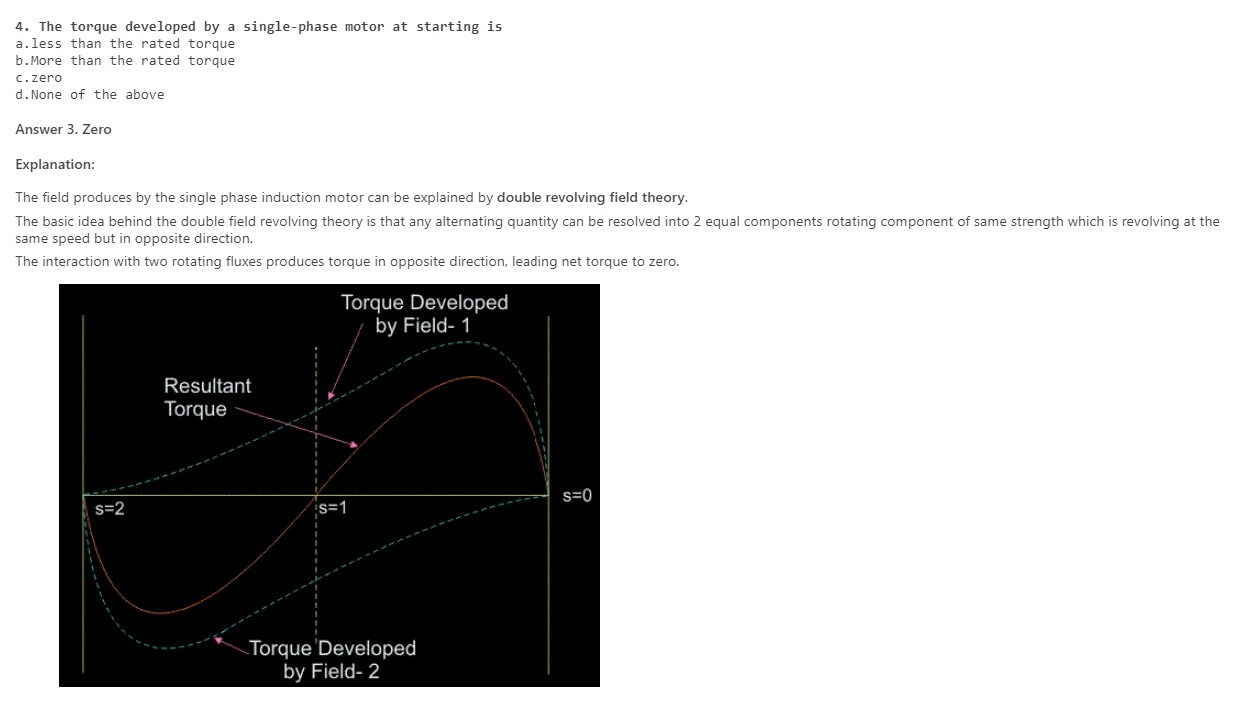5. In a capacitor start and run motors the function of the running capacitor in series with the auxiliary winding is to
a.Improve power factor
b.Reduce fluctuations in torque
d.To improve torque```

Explanation:

• Inductive Loads consume Reactive Power and Capacitive Loads deliver Reactive Power.
• The Power Factor Angle is inversely proportional to the Power Factor( reducing the angle gap between the Voltage vector and the Current Vector improved power factor).
• So in a single phase Induction Motor, a Capacitor Bank is connected, than the Reactive power demand by the Inductive load (Induction Motor) is supplied by the Capacitor Bank, not from the Source.
• Therefore the system reactive power demand is reduced and the Power factor is improved.
```6. Which of the following motor will have relatively higher power factor?
a.Capacitor start motor
c.Capacitor run motor
d.Split phase motor```
```Answer 3. Capacitor run motor
Explanation:```
• `In capacitor run motor the starting winding remains connected all the times. There is no centrifugal switch in the capacitor run motor.`
• `Because the capacitor remains in the circuit all the time a lower value capacitor is used the lower value capacitor has more phase shift as compared to the high-value capacitor, therefore, winding current is low.`
`Therefore the capacitor run motor has high running torque and improved power factor.`
```7. A centrifugal switch is used to disconnect ‘starting winding when motor has
a.Picked up 10% speed
b.Picked up 20% speed
c.Picked up 5 – 10% speed
d.Picked up 50 – 70% speed```
```Answer 4. Picked up 50% – 70% speed
Explanation:```
`The centrifugal switch is used to disconnect the starting winding of the motor once the motor approaches its normal operating speed i.e 50% – 70% speed.`

8. In repulsion motor direction of rotation of motor
a.Same as that of brush shift
b.Independent of brush shift
c.Opposite to that of brush shift
d.None of the above
Answer 1. Same as that of brush shiftExplanation:

• The direction of rotation is determined by the position of the brushes with respect to the magnetic field of the stator.
• If the brushes are shifted clockwise from the main magnetic axis, the motor will rotate in a clockwise direction.
• If the brushes are shifted counterclockwise from the main magnetic axis, the motor will rotate in a counter-clockwise direction
```10. Which of the following motor is used in the mixer?
a.Repulsion Motor
b.Reluctance Motor
c.Hysteresis Motor
d.Universal Motor```

Explanation:

• We use universal motors in mixer grinders. Universal motors can run both on a.c. and d.c.
• The universal motor works on the same principle that DC series motor works. DC series motor has the characteristics of operating at high-speed when there is no load and operating at low-speed when the load is applied. It has high starting torque characteristics.
• So it is used in mixers, where initially we put some load at starting.
Python Programming for Complete Beginners

11. The rotor of a three phase induction motor can never attain synchronous speed.
a) True
b) False

Explanation: Because then there will be no torque developed and flux cutting.

12. The direction of rmf when a single phase supply is given to stator of three phase induction motor is?
a) Zero
b) Ns
c) 2Ns
d) -Ns

Explanation: There will be no rmf generated as a single phase is only fed to the three phase of induction motor.

13. The direction of rmf when a three phase supply is given to stator of three phase induction motor is?
a) Zero
b) Ns
c) 2Ns
d) -Ns

Explanation: There will be rotating magnetic field will be produced causing the emf to be induced and the current will flow in the rotor at a speed of synchronous speed.

14. Two three phase induction motors A and B are identical in all respects except that motor A has a larger air-gap than motor B. Which motor will have more no load current?
a) A
b) B
c) Both A and B
d) Neither A nor B

Explanation: As the air gap of motor A is more, it will require more flux to excitation and so the no load current.

15. Two three phase induction motors A and B are identical in all respects except that motor A has a larger air-gap than motor B. Which motor will have poorer power factor?
a) A
b) B
c) Both A and B
d) Neither A nor B

Explanation: Due to larger air gap of motor A, it will need more of exciting current. So the no load angle will increase and power factor will deteriorate.

16. Two three phase induction motors A and B are identical in all respects except that motor A has a larger air-gap than motor B. Which motor will have better full-load power factor?
a) A
b) B
c) Both A and B
d) Neither A nor B

Explanation: At full load conditions, the excitation current is not very high and so the power factor improves.

17. A three phase induction motor is sometimes calles a generalized transformer in so far as voltage and frequency transformation has been concerned. How a three phase induction motor operates when rotor frequency is equal to stator frequency?
a) It will not operate
b) It will operate as induction motor only
c) It will operate as induction generator
d) It will operate in braking mode
Explanation: As the rotor and stator frequencies are equal, there will be no slip and so the zero emf will be produced.

Get Engineering Viva Question with Answers

18. A three phase induction motor is sometimes called a generalized transformer in so far as voltage and frequency transformation has been concerned. How a three phase induction motor operates when rotor frequency is greater than stator frequency?
a) Rotor will be driven against rmf
b) Rotor will be driven in direction of rmf
c) No emf will be induced
d) The losses will be maximum

Explanation: As the rotor rotates at larger speed than the stator, the rmf speed is less than the rotor speed and it tries to rotate in the opposite direction to rmf.

19. A three phase induction motor is sometimes called a generalized transformer in so far as voltage and frequency transformation has been concerned. How a three phase induction motor operates when rotor frequency is less than stator frequency?
a) It operates as induction motor
b) Emf is induced in rotor
c) Rmf will rotate at synchronous speed
d) All of the mentioned

Explanation: It will operate as induction motor.

20. A three phase induction motor is sometimes called a generalized transformer in so far as voltage and frequency transformation has been concerned. Then the rotor emf E2 and the rotor current I2 are zero at _________
a) Ns
b) 2Ns
c) Zero
d) Slip of 50%

Explanation: The rotor emf and current are zero at the speed of synchronous speed of the machine.
Python Programming for Complete Beginners

```I. stator copper loss
II. stator core loss
III. rotor core loss
IV. friction and windage losses
V. Rotor copper loss
```

a) I, II, IV
b) II, III, IV
c) I, III, II, IV
d) II, IV

Explanation: Blocked rotor test will give stator losses and the friction and windage losses.

22. If use auto transformer method starting to start an induction motor to replace star-delta method, then the required tapping on the transformer will be _________
a) 57.73%
b) 86.7%
c) 57%
d) 66.66%

Explanation: k = 1/1.73 = 57.73%.

23. During the blocked rotor test the wattmeter reading will be _________

```I. stator copper loss
II. stator core loss
III. rotor core loss
IV. friction and windage losses
V. Rotor copper loss
```

a) I, V
b) II, III, IV
c) I, III, II, IV
d) II, IV

Explanation: Blocked rotor test will account for copper losses.

24. The starting method for a 3-phase squirrel-cage induction motor which is inferior in view of poor starting torque per ampere of line current drawn is?
a) series-inductor method of starting
b) direct-on-line starting
c) auto-transformer method
d) star-delta method

Explanation: Due to inductor method, reactance increases and the starting torque decreases.

25. Reactor method of starting is preferred over resistance method because _________

```I. power factor improves
II. increase starting torque
III. lower losses
IV. more effective in reducing voltage
```

a) III, IV
b) I, II, III
c) II, III, IV
d) I, I

Explanation: By reactor method of starting, losses will be lesser and voltage reduction is effective than the resistance method of starting.

26. How many steps are involved in the construction of a single-phase induction motor?
a) 3
b) 4
c) 5
d) 6

Explanation: There are 5 steps in the construction of the single-phase induction motor. They are stator, stator windings, rotor, starting switches, electrolytic capacitor.

27. What is the lamination used for the stator?
a) cast iron
b) die cast aluminium alloy frame
c) cast iron or die-cast aluminium alloy frame
d) cast iron and die-cast aluminium alloy frame

Explanation: The stator is made up of a block of laminations. The block of laminations are made up of cast iron or die-cast aluminium alloy frame.

28. What type of coils are used for winding the single-phase induction motor generally?
a) rectangular coils
b) square coils
c) cruciform coils
d) circular coils

Explanation: The slots house the starting and running windings. The single phase induction motors are generally wound with concentric coils.

29. How many kinds of single phase windings are present?
a) 2
b) 3
c) 4
d) 5

Explanation: There are basically 3 kinds of single phase windings. They are concentric, progressive and skein.

30. How are the poles and pitches in the concentric windings?
a) single pole, different pitches
b) different pole, different pitches
c) different pole, single pitch
d) single pole, single pitch

Explanation: The concentric windings have a single pole for a common centre. They have different pitches for each individual coil.
Python Programming for Complete Beginners

31. What is the form of the progressive windings?
a) double layer diamond coil windings
b) single layer diamond coil windings
c) multi layer diamond coil windings
d) three layer diamond coil windings

Explanation: The progressive windings is one kind of the stator windings. They are in the form of the single layer diamond coil windings.

32. When is the skein winding made use of?
a) when small amount of relatively small size wire is used
b) when large amount of relatively small size wire is used
c) when large amount of relatively large size wire is used
d) when small amount of relatively large size wire is used

Explanation: Skein winding is one of the 3 kinds of single phase windings used. It is used when small amount of relatively small size wire is used.

32. What kind of motor employs the skein winding made use of?
a) maximum horse power single phase induction motor
b) fractional horse power single phase induction motor
c) minimum horse power single phase induction motor
d) zero horse power single phase induction motor

Explanation: The skein winding is one of the 3 kinds of single phase induction motor. The skein winding is used when fractional horse power single phase induction motor is used.

33. Which winding is mostly used winding in the single phase induction motor?
a) circular winding
b) concentric winding
c) progressive winding
d) skein winding

Explanation: The concentric winding is the most widely used winding. It is also the most flexible winding of the windings used in the single phase induction motor.

35. What is/are the advantages of the skein winding?
a) low cost to wind
b) low cost to insert
c) permits some freedom of choice of distribution
d) low cost to wind, low cost to insert, permits some freedom of choice of distribution

Explanation: The skein winding is the low cost to wind and to insert. It also permits some freedom of choice of distribution.

37. What material is used in the tunnel of the rotor of the single phase induction motor?
a) aluminium
b) copper
c) steel
d) wood

Explanation: The rotor consists of a block of slotted laminations. The slots form a series of tunnels that are filled with aluminium in its molten state.

38. What type of operations are used in the starting switches?
a) mechanical operation
b) electrical operation
c) centrifugal operation and mechanical operation
d) centrifugal operation

Explanation: The starting switch is used to cut the auxillary winding when the motor attains 75% of the full load speed. The switches operate in both the centrifugal as well as mechanical operation.

39. The ac electrolytic capacitor is formed by winding two sheets of etched aluminium foil.
a) true
b) false

Explanation: Modern capacitor start motors employ ac electrolytic capacitors. The ac electrolytic capacitor is formed by winding two sheets of etched aluminium foil, separated by two layers of insulating paper, into a cylindrical shape.

40. The electrolytic capacitor and insulator unit is impregnated using ethylene glycol or a derivative.
a) true
b) false